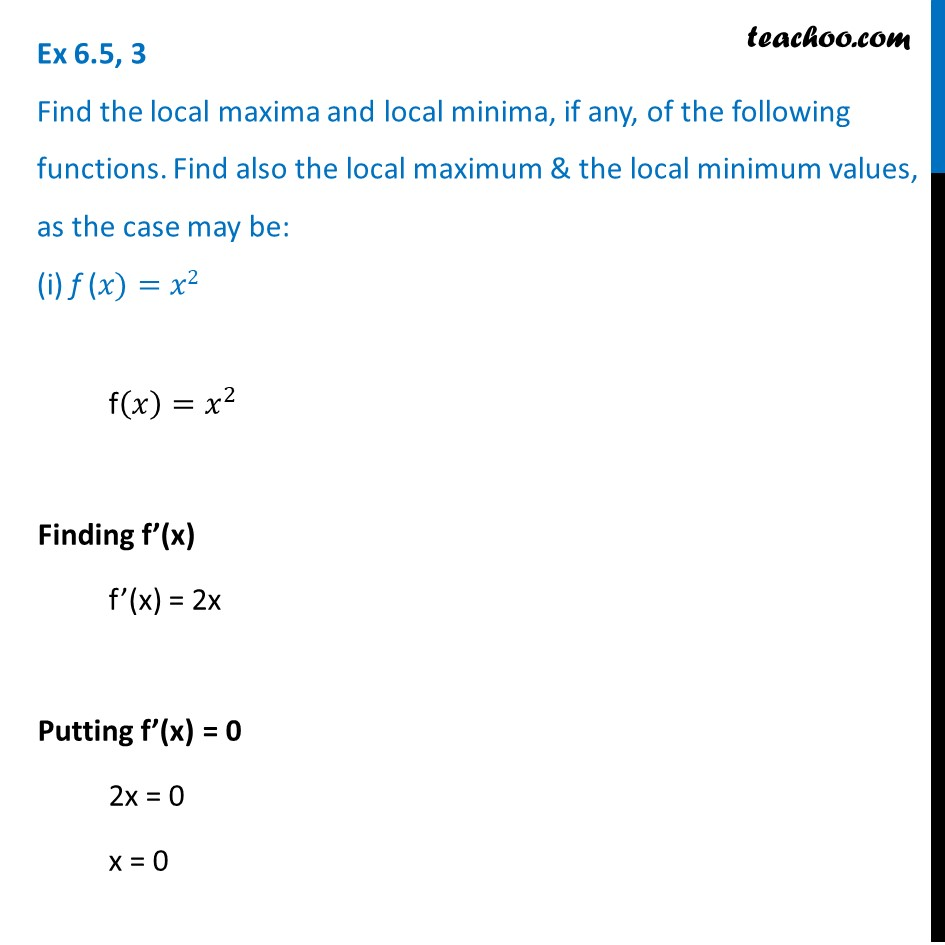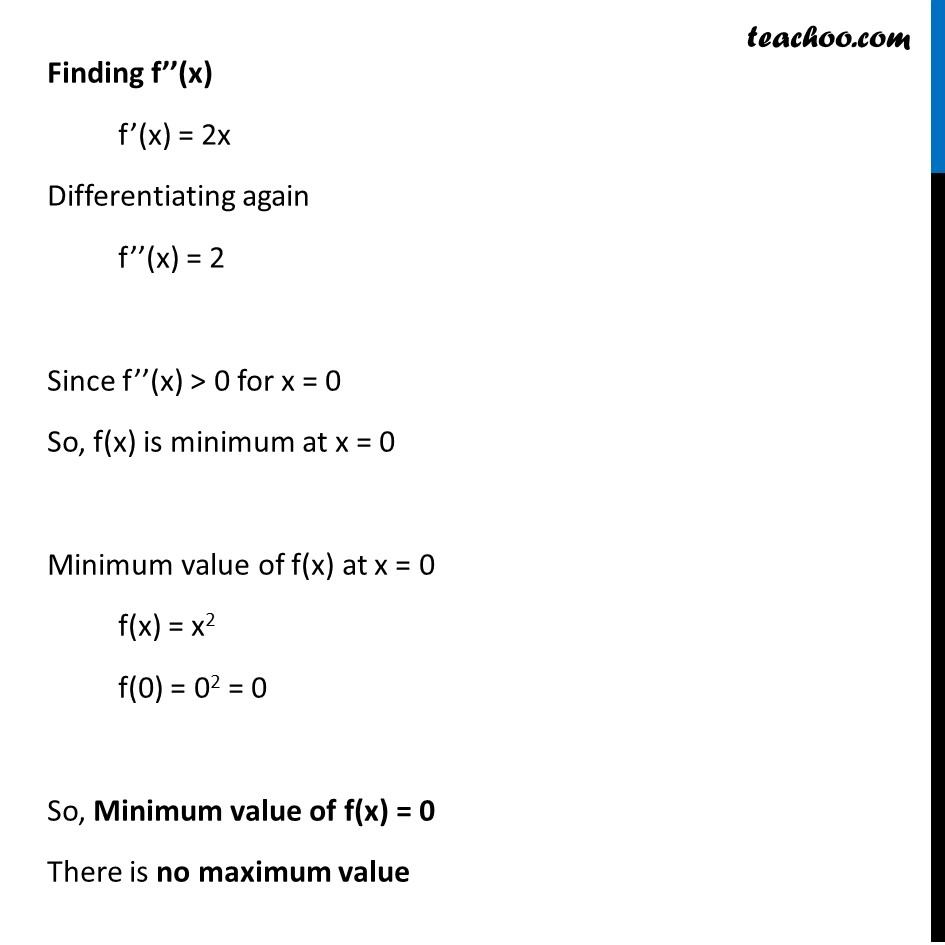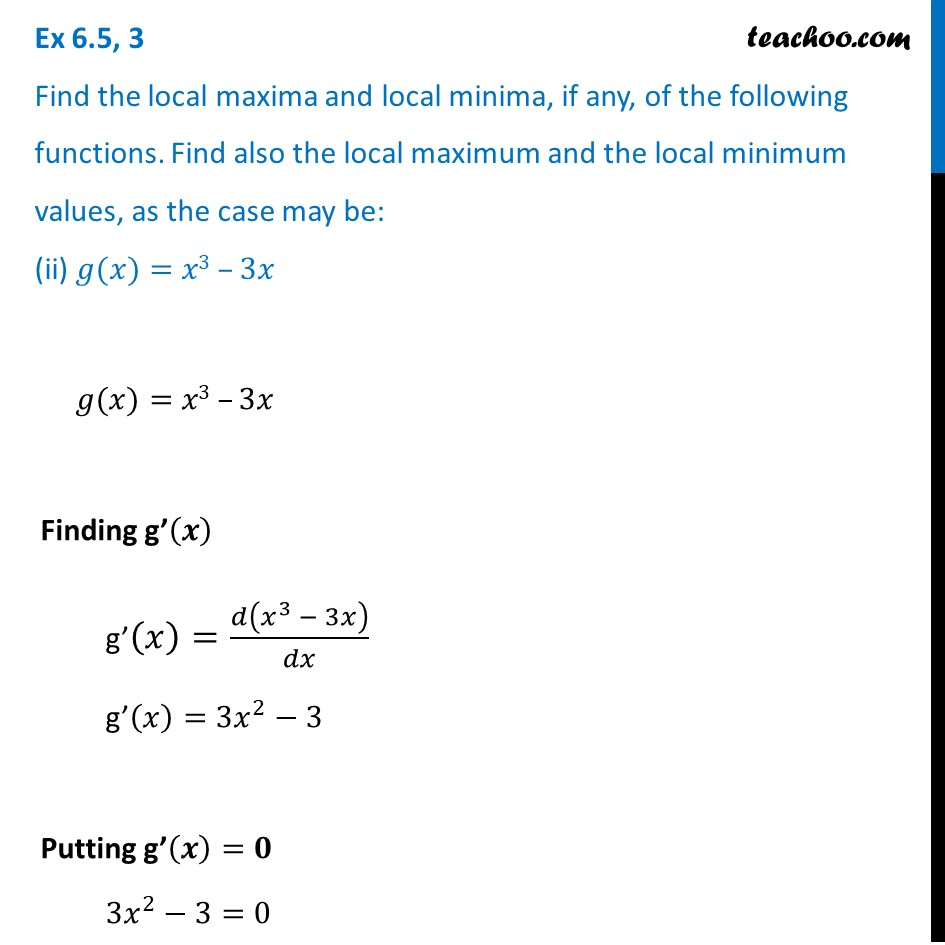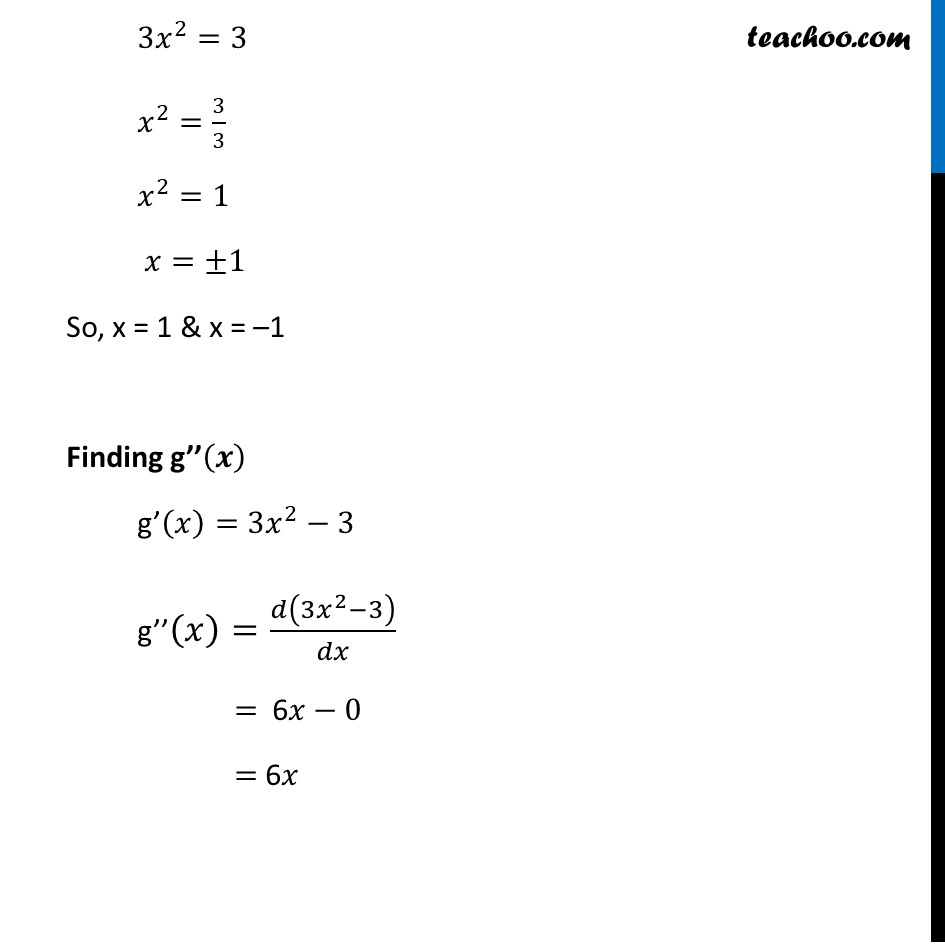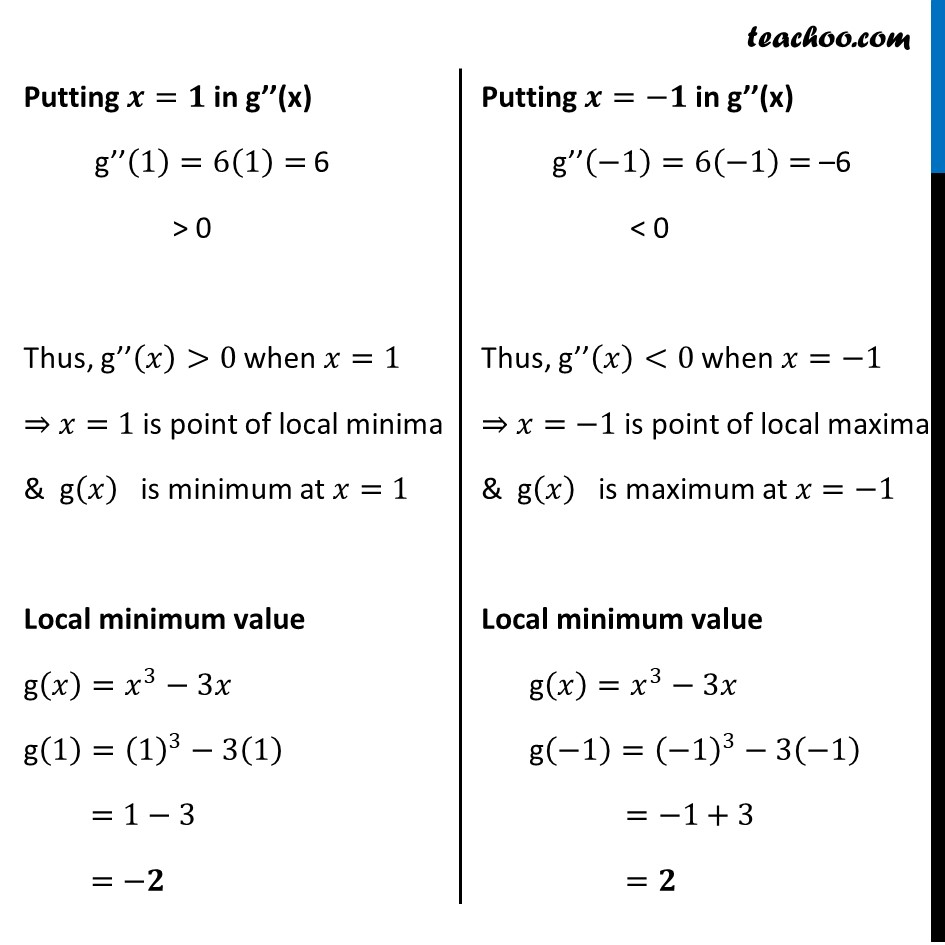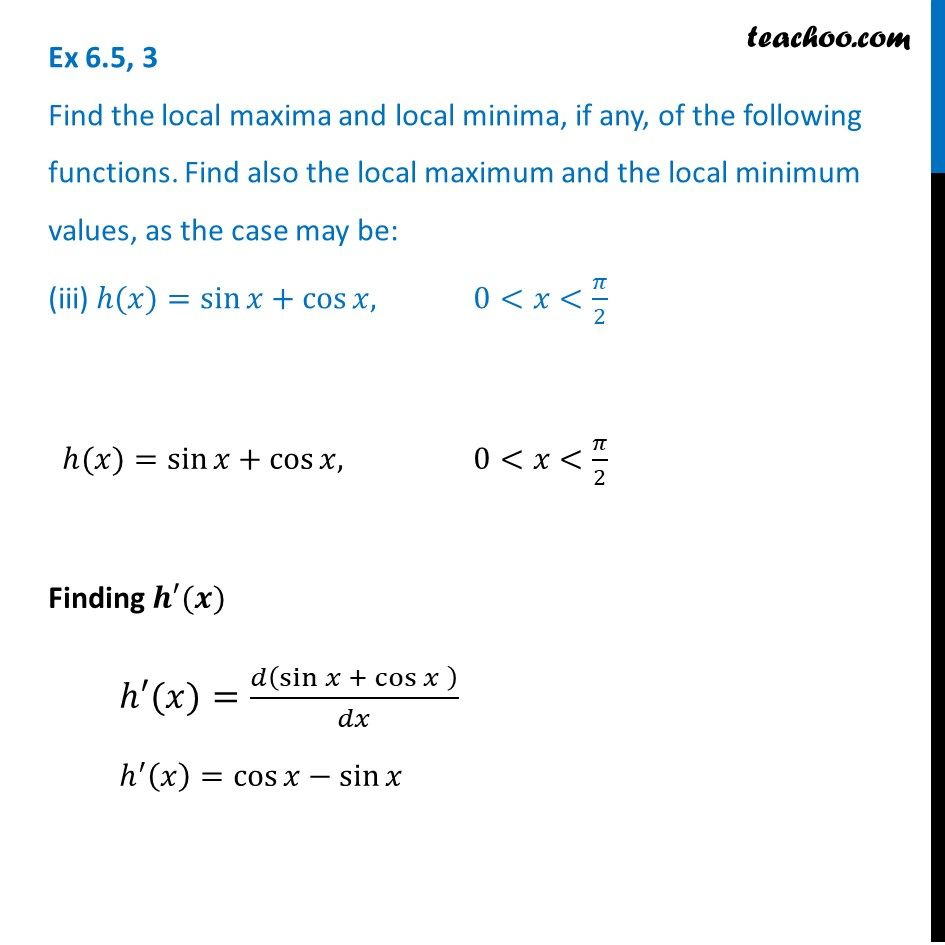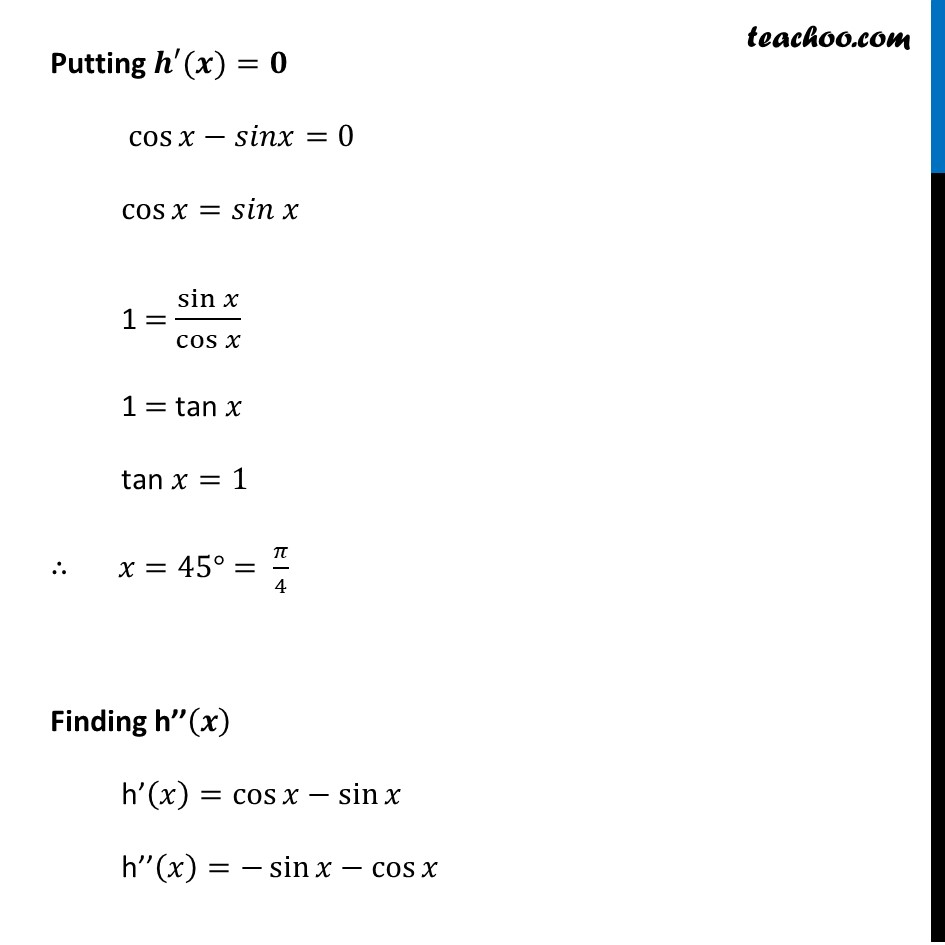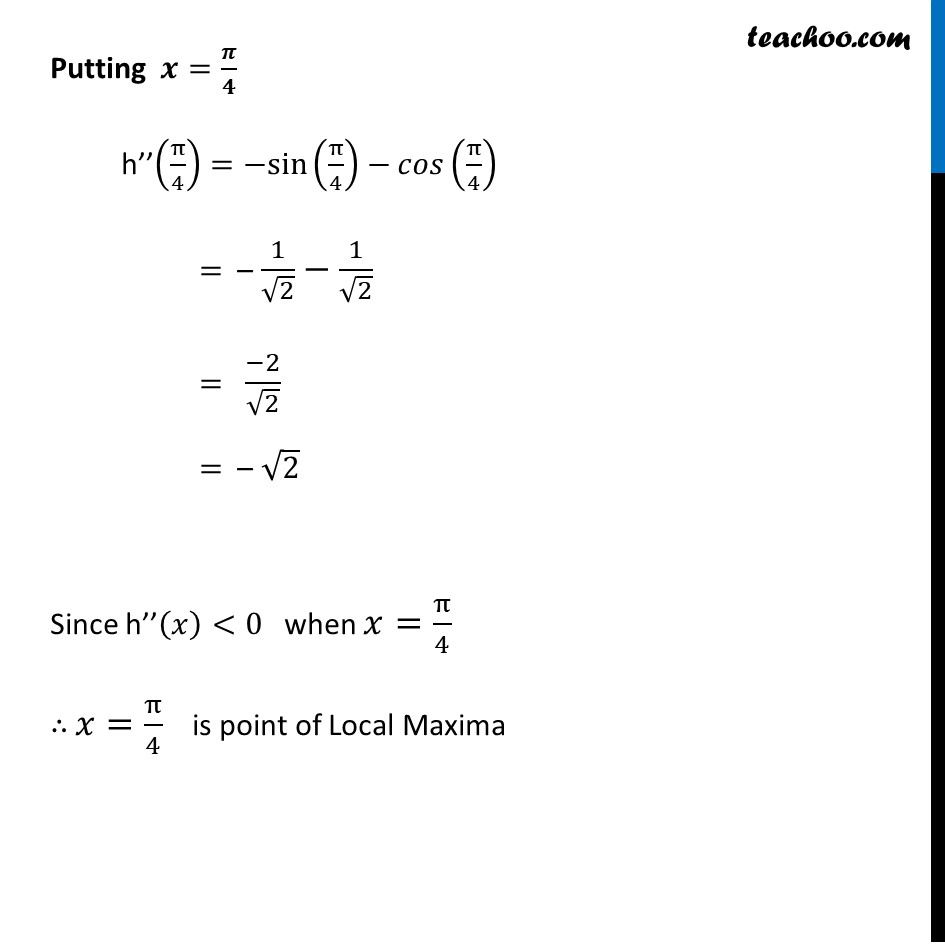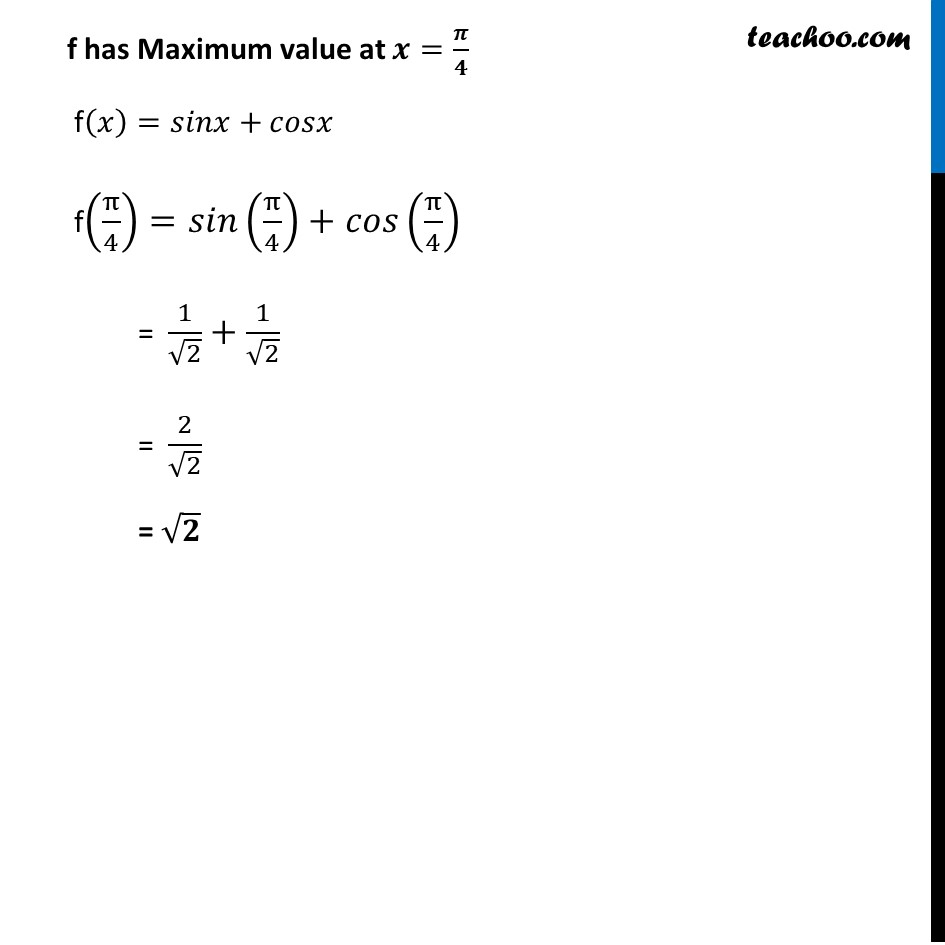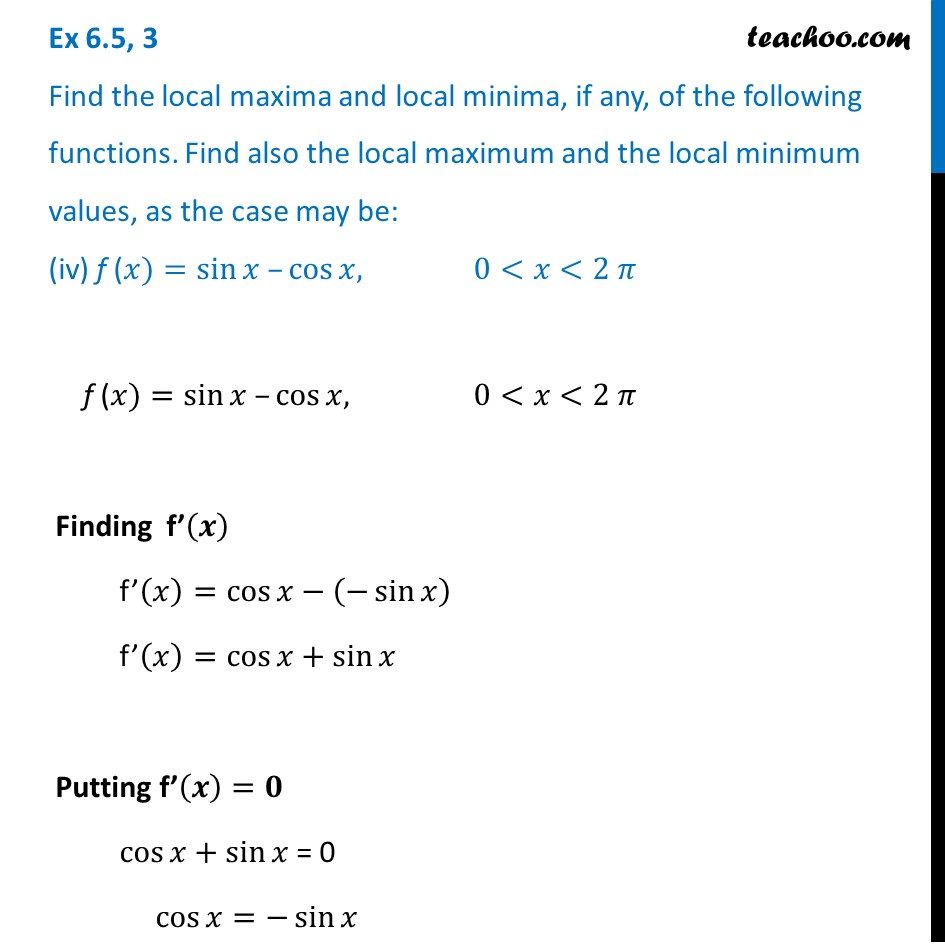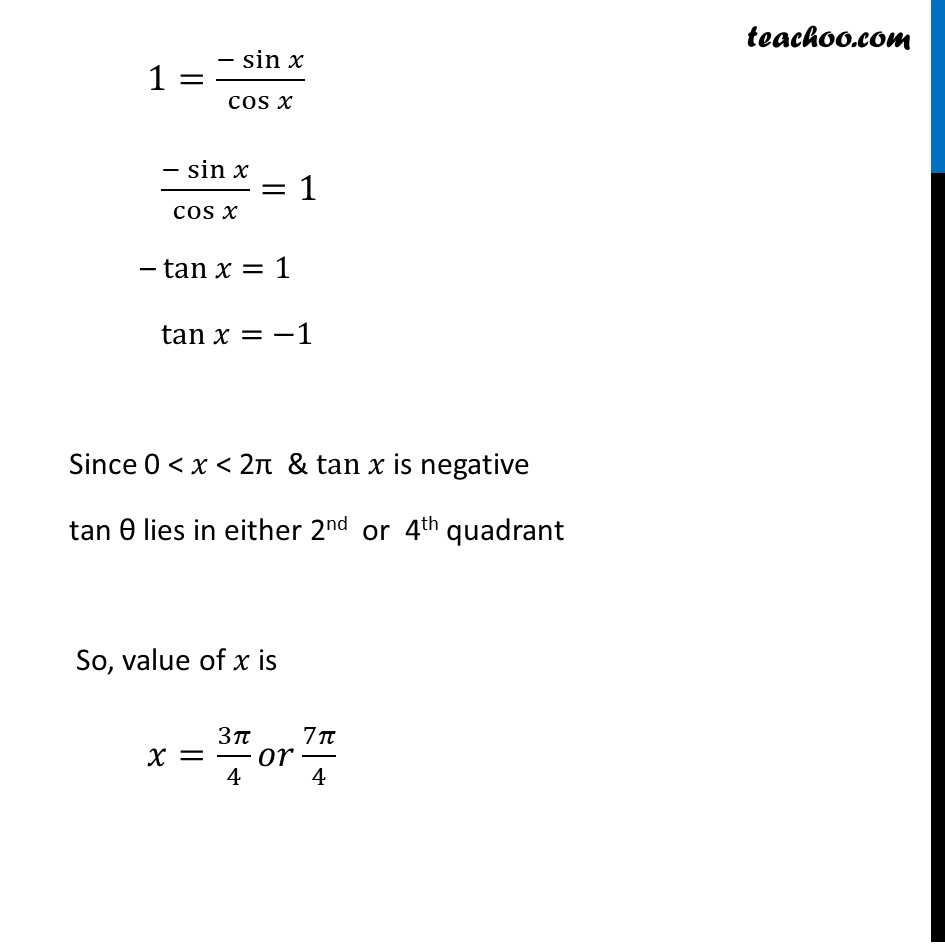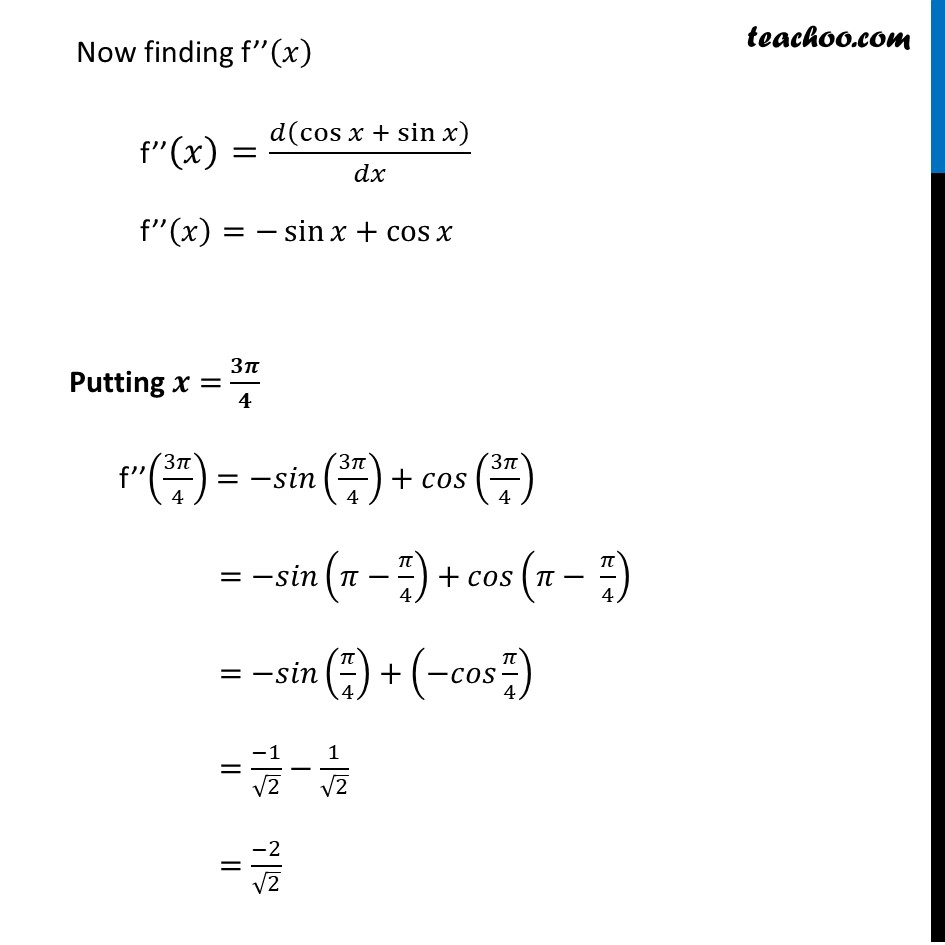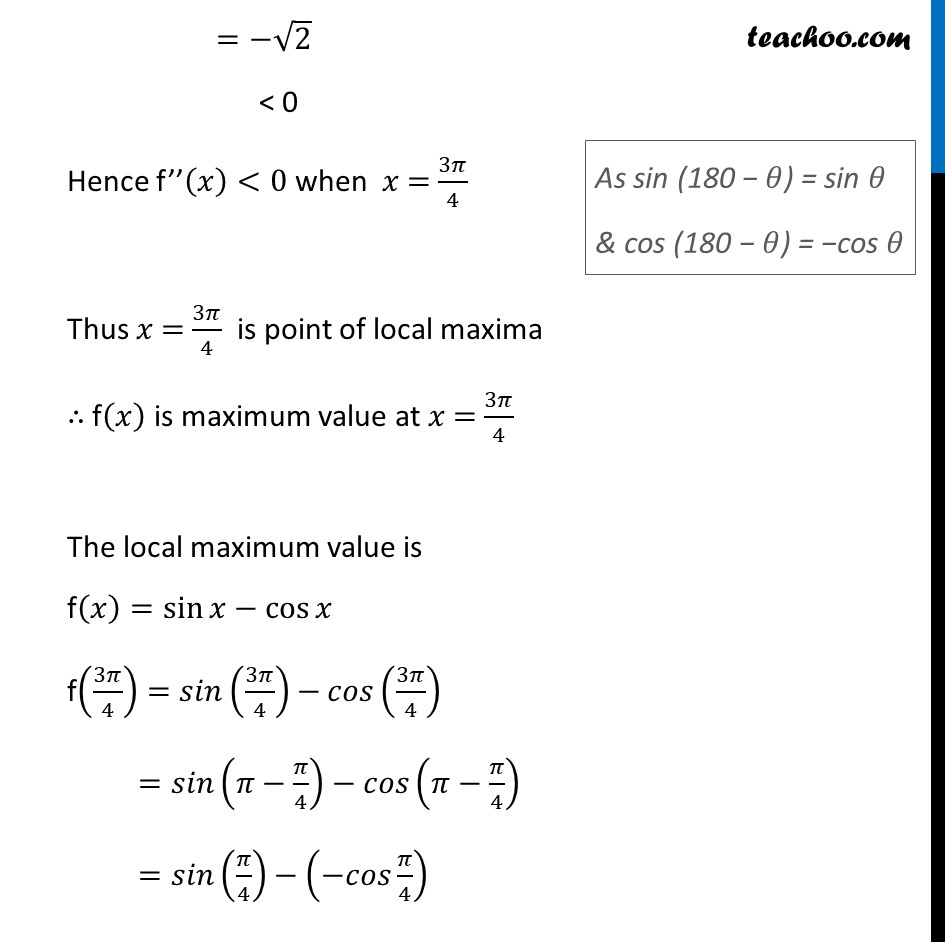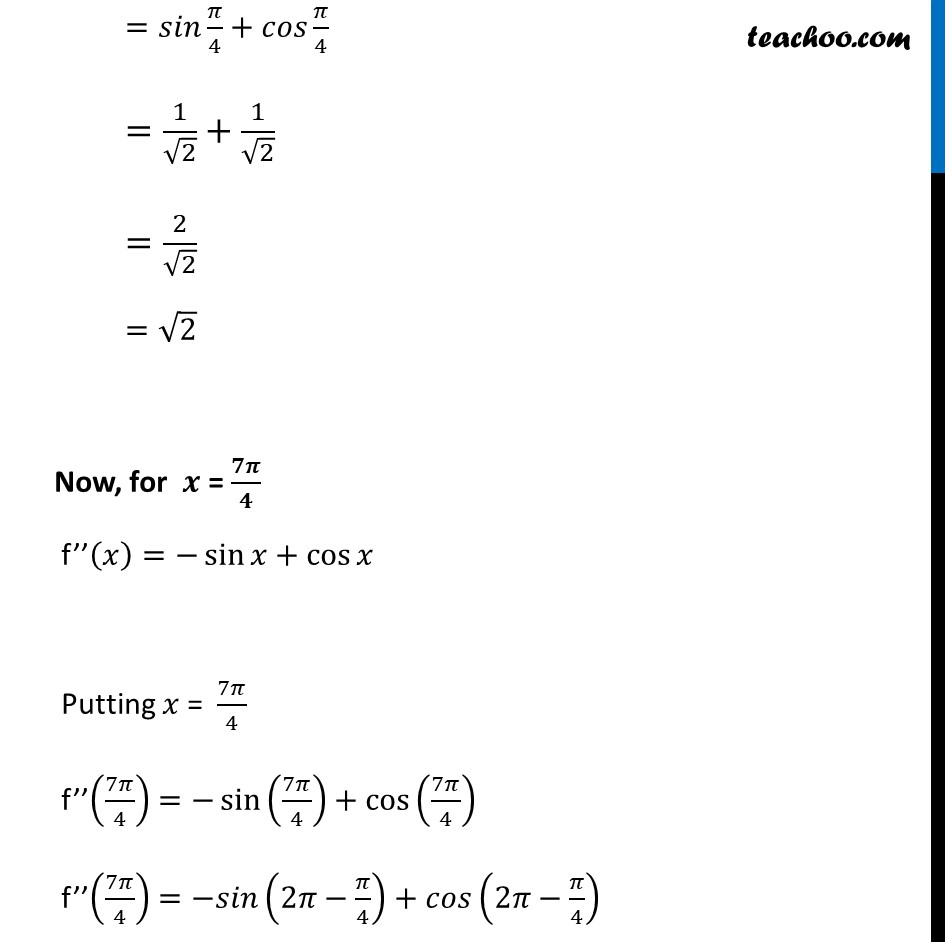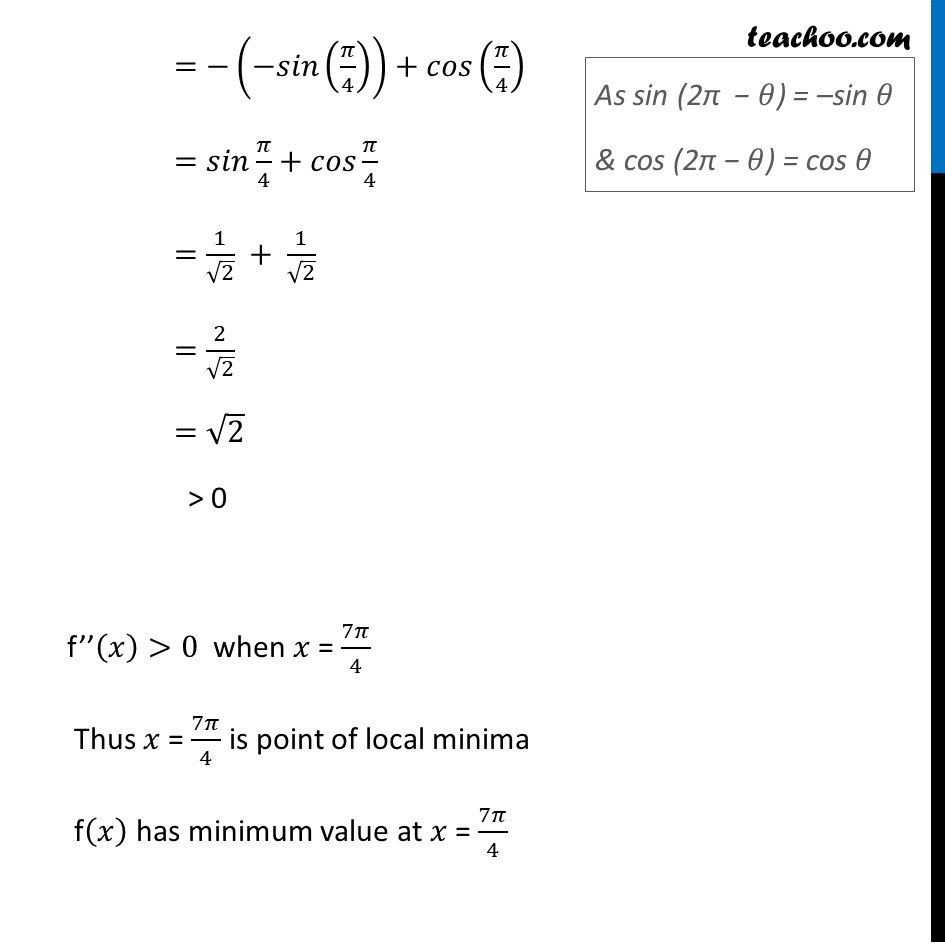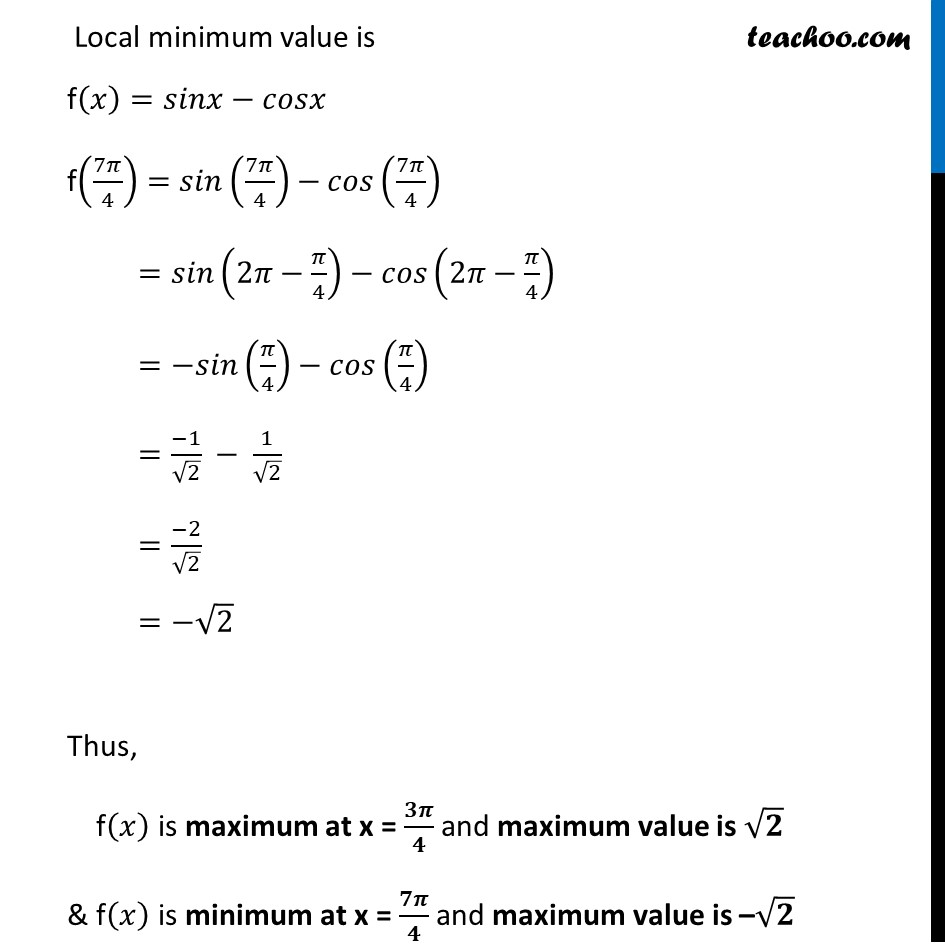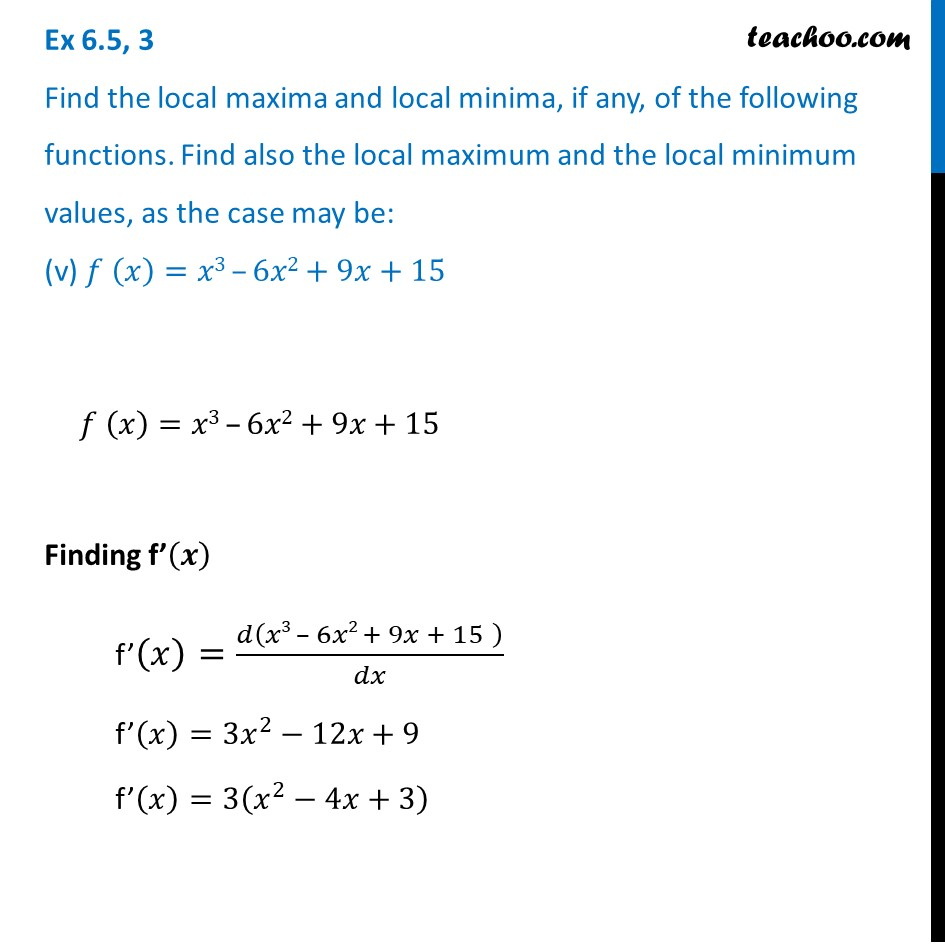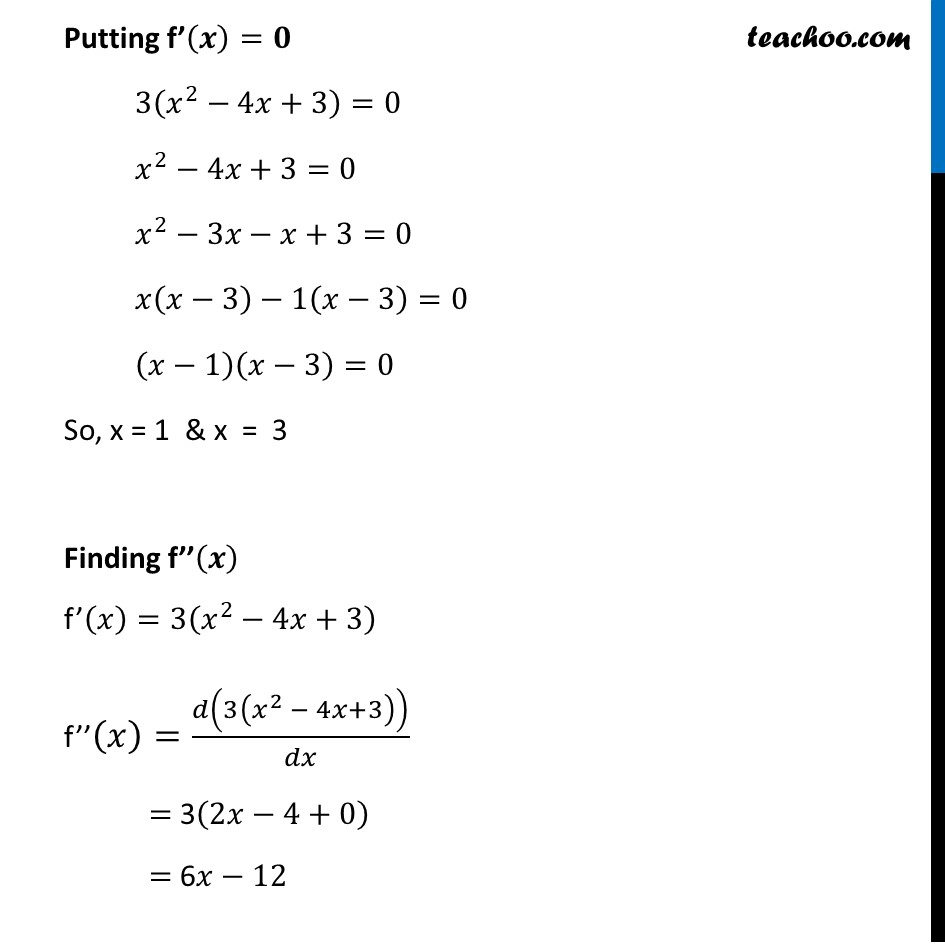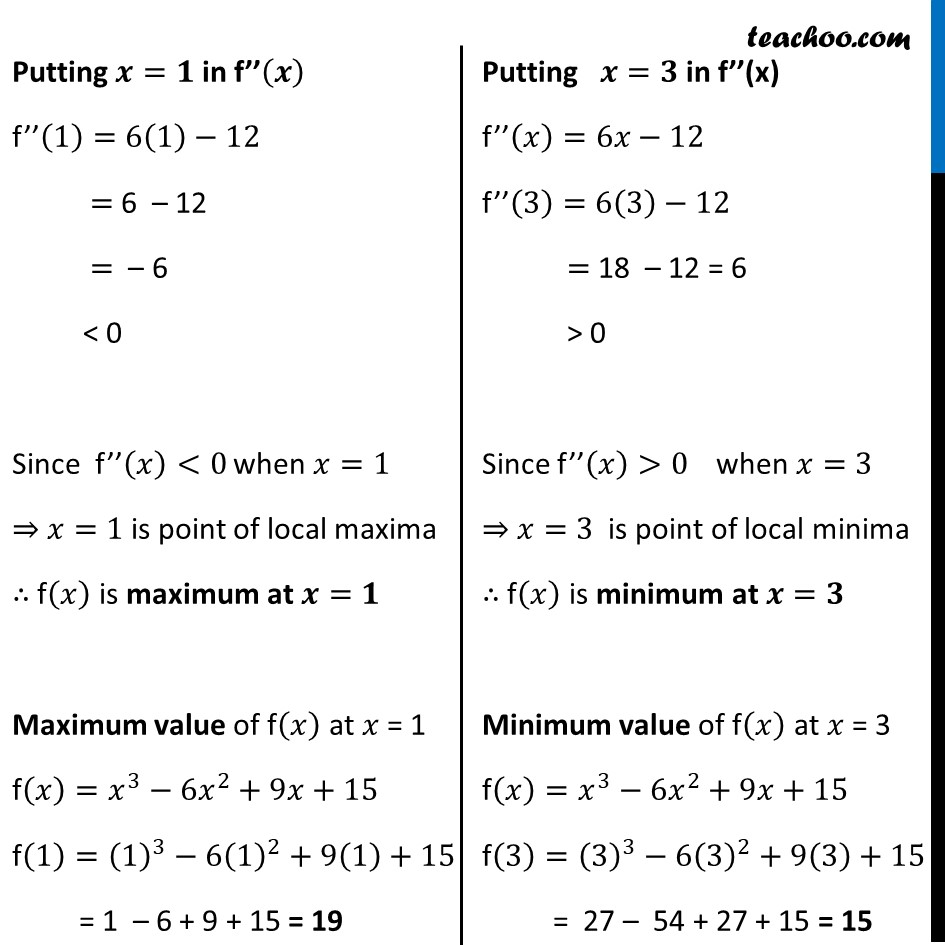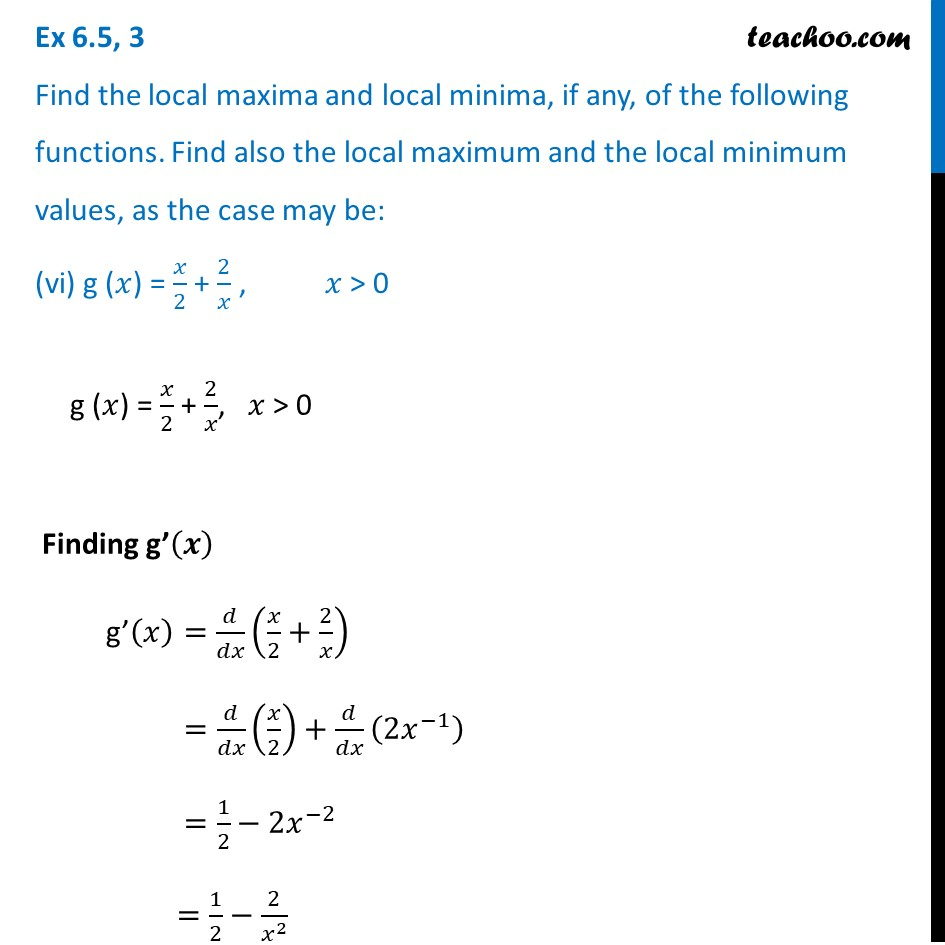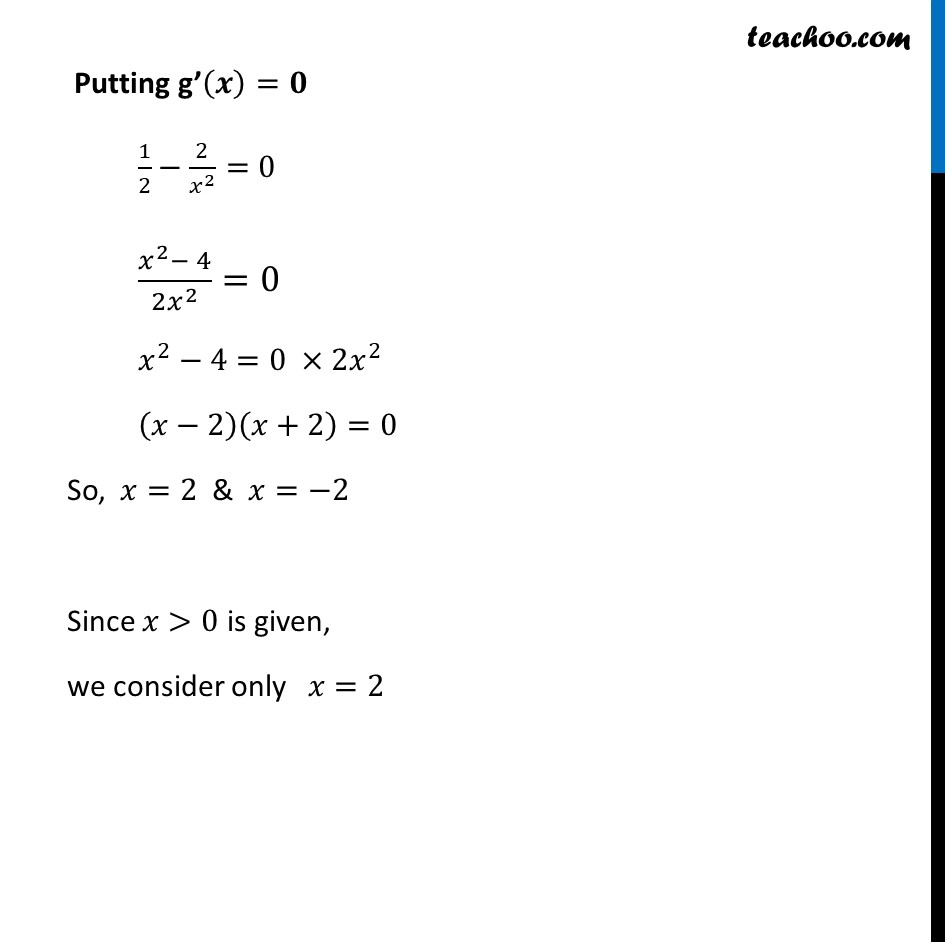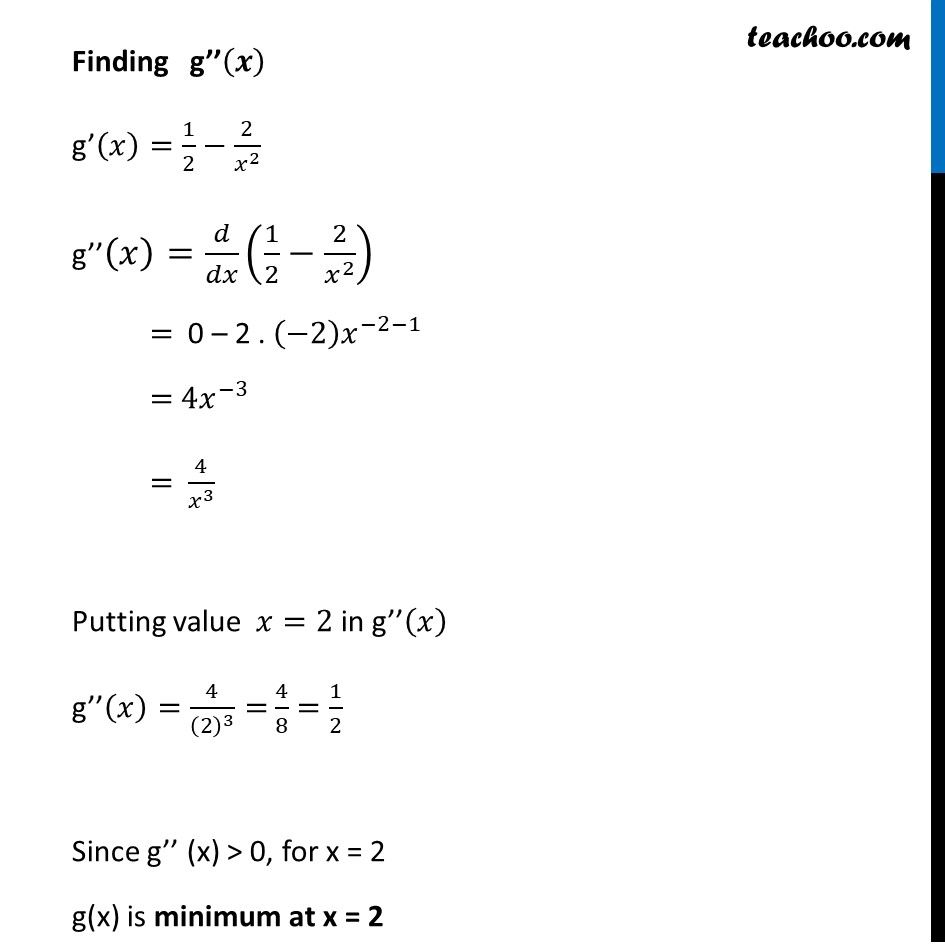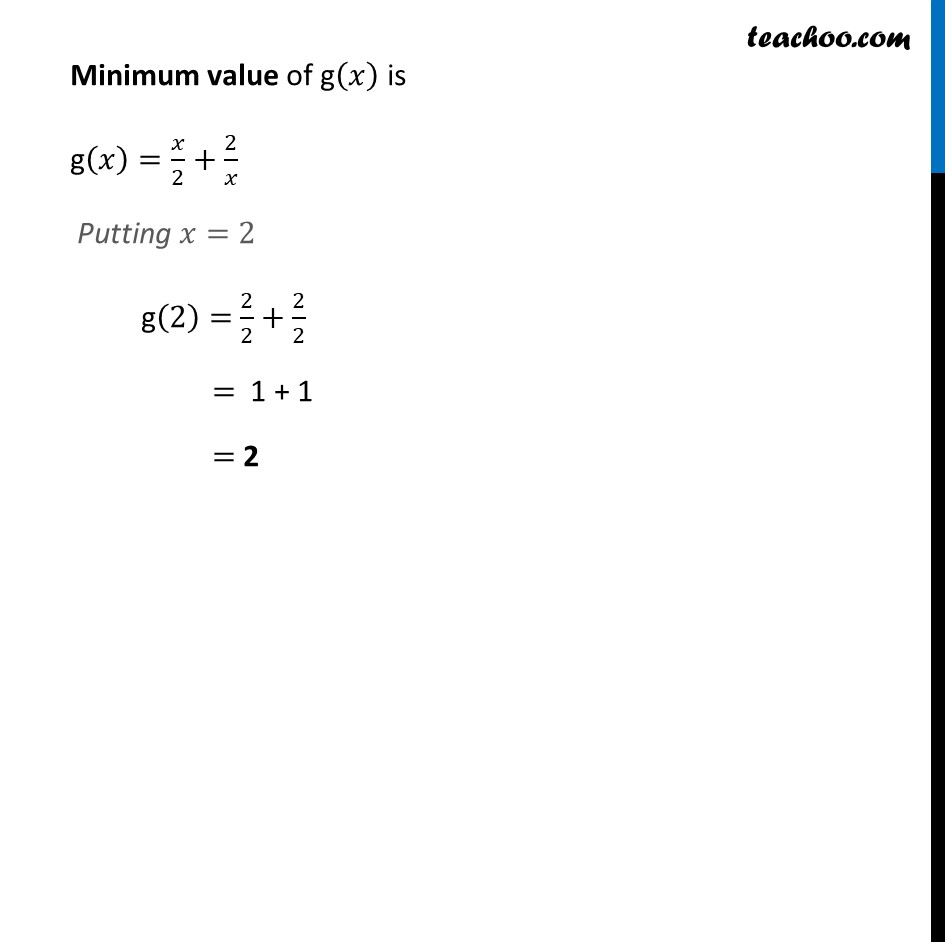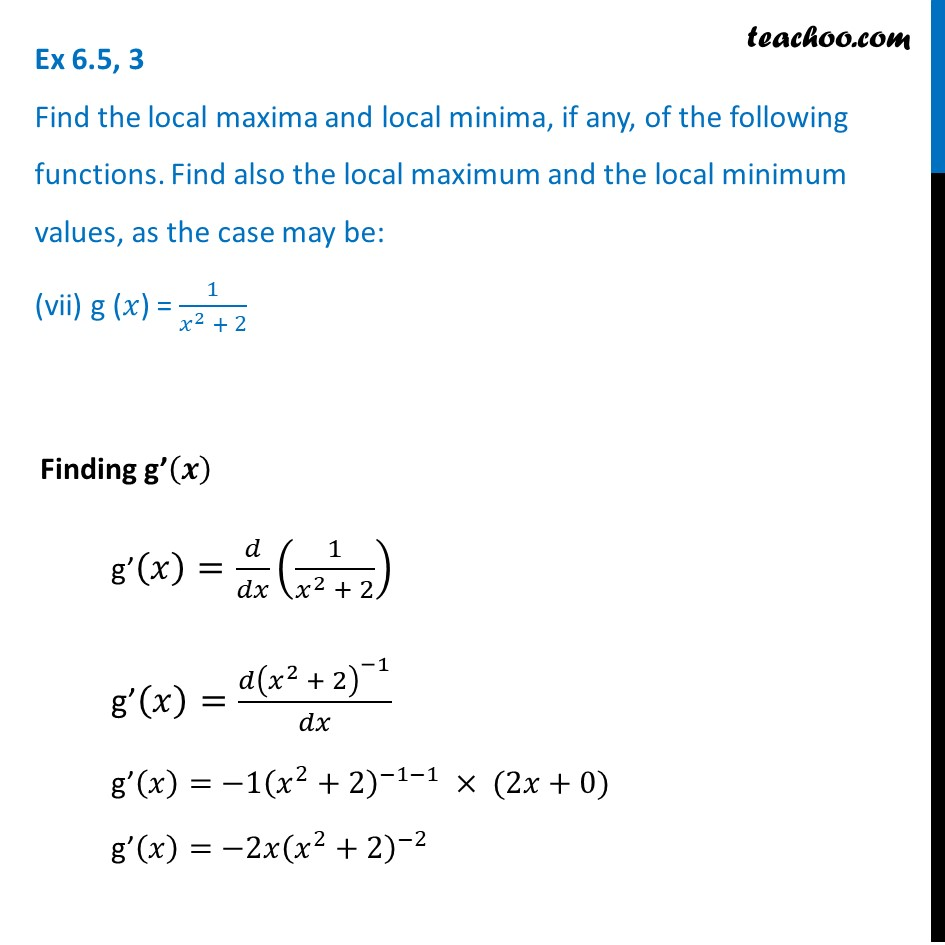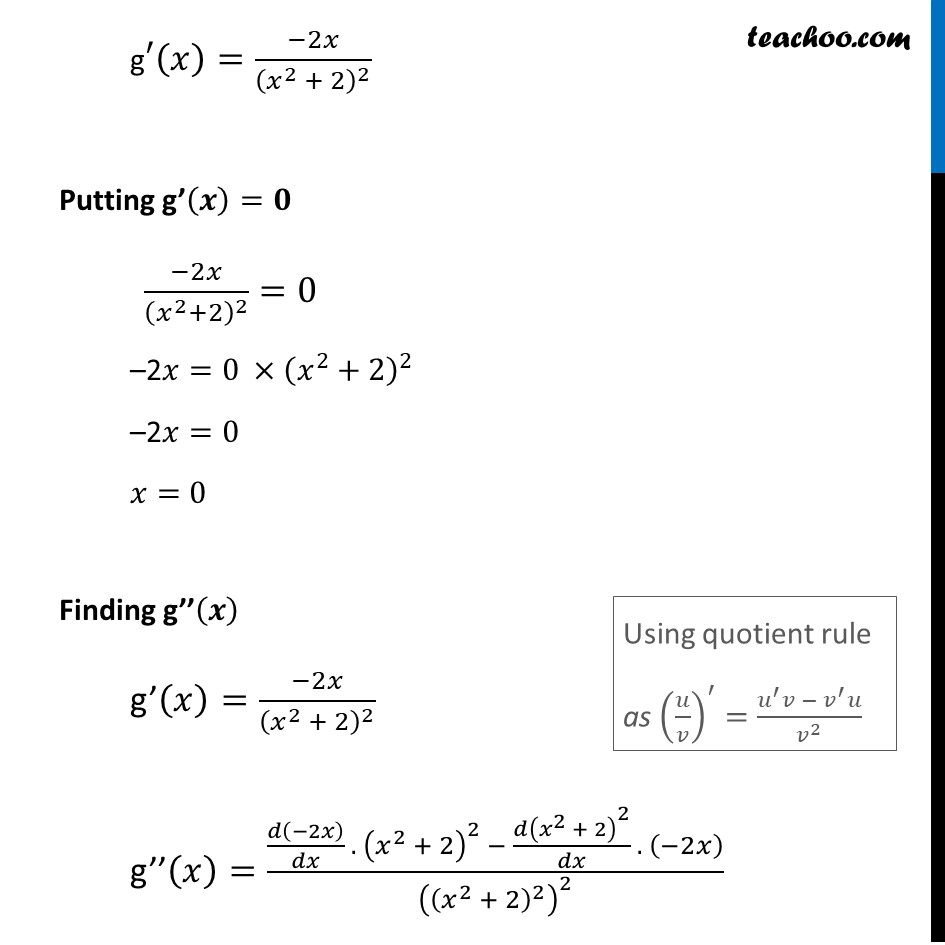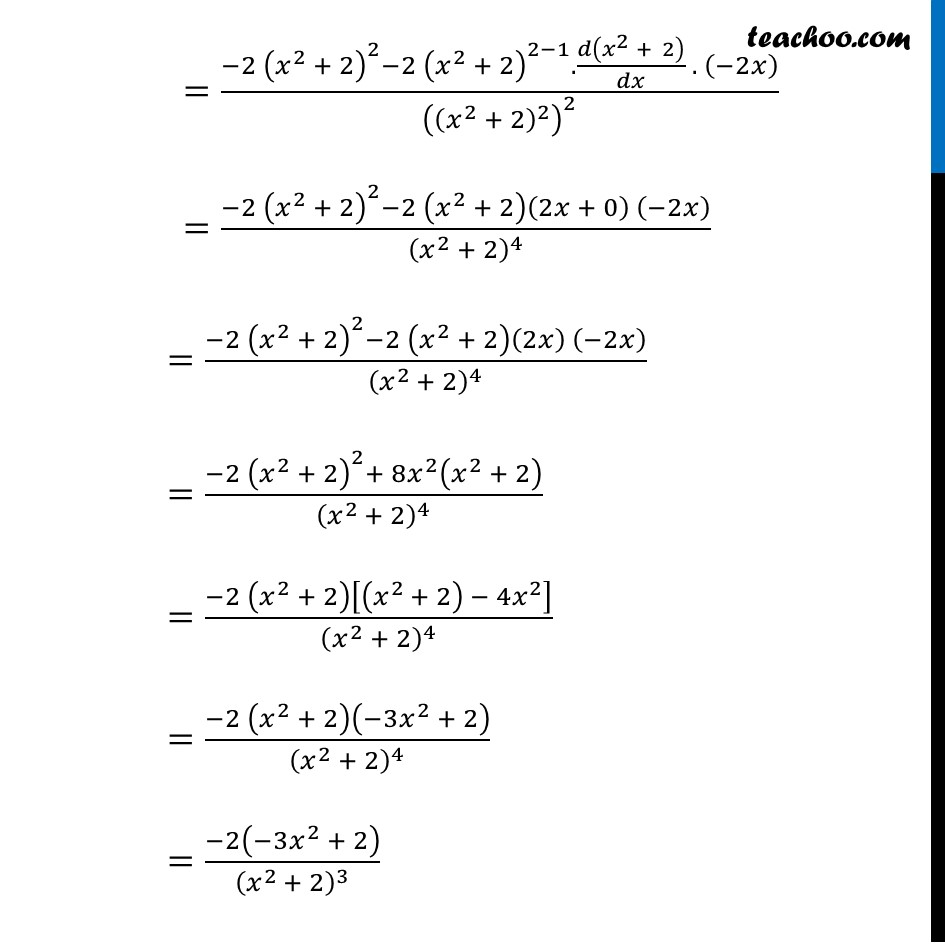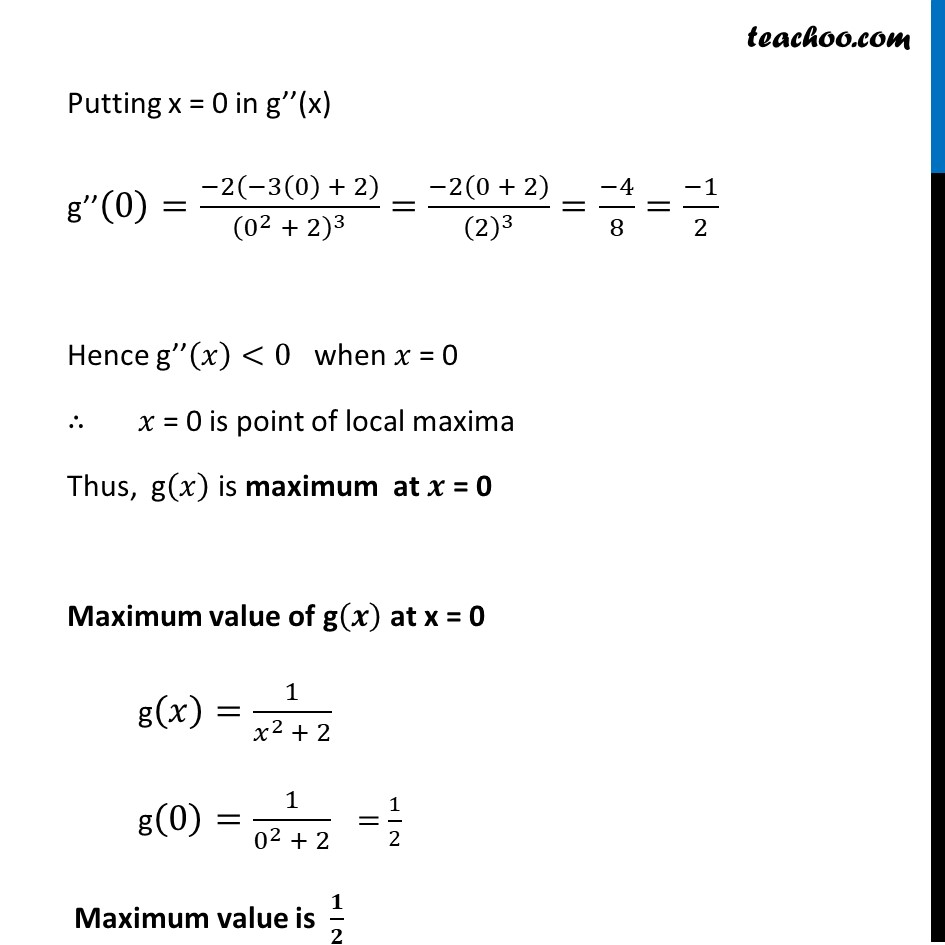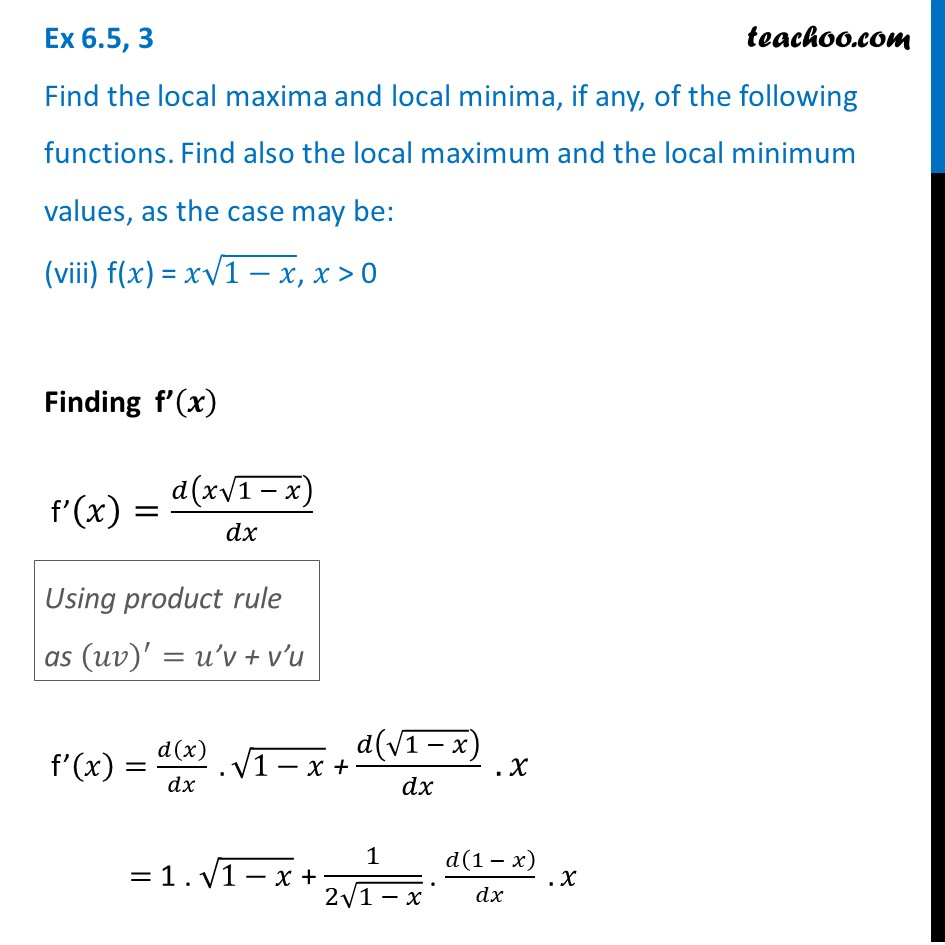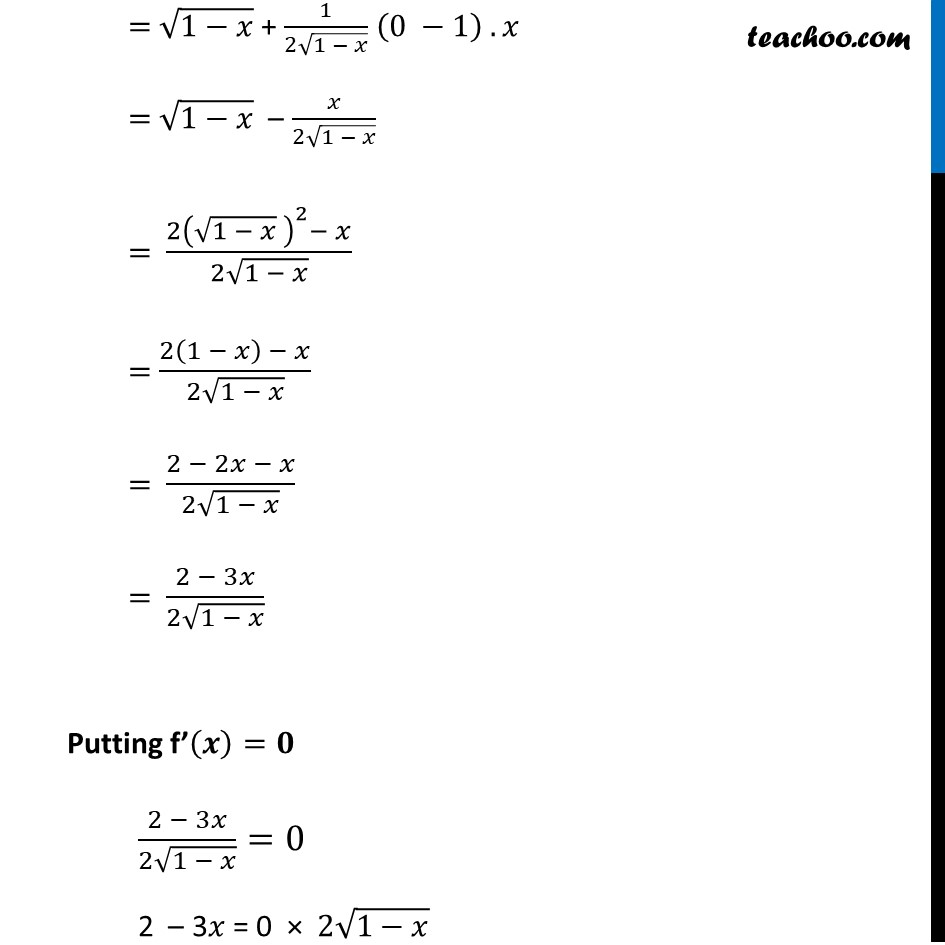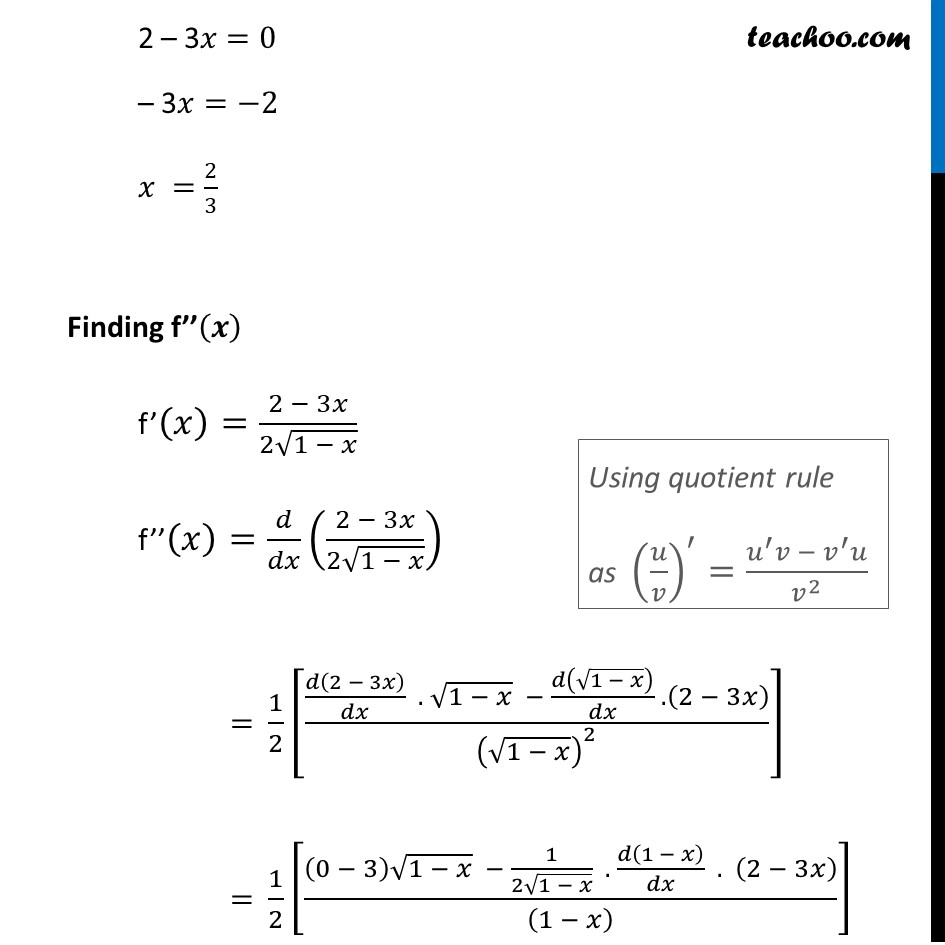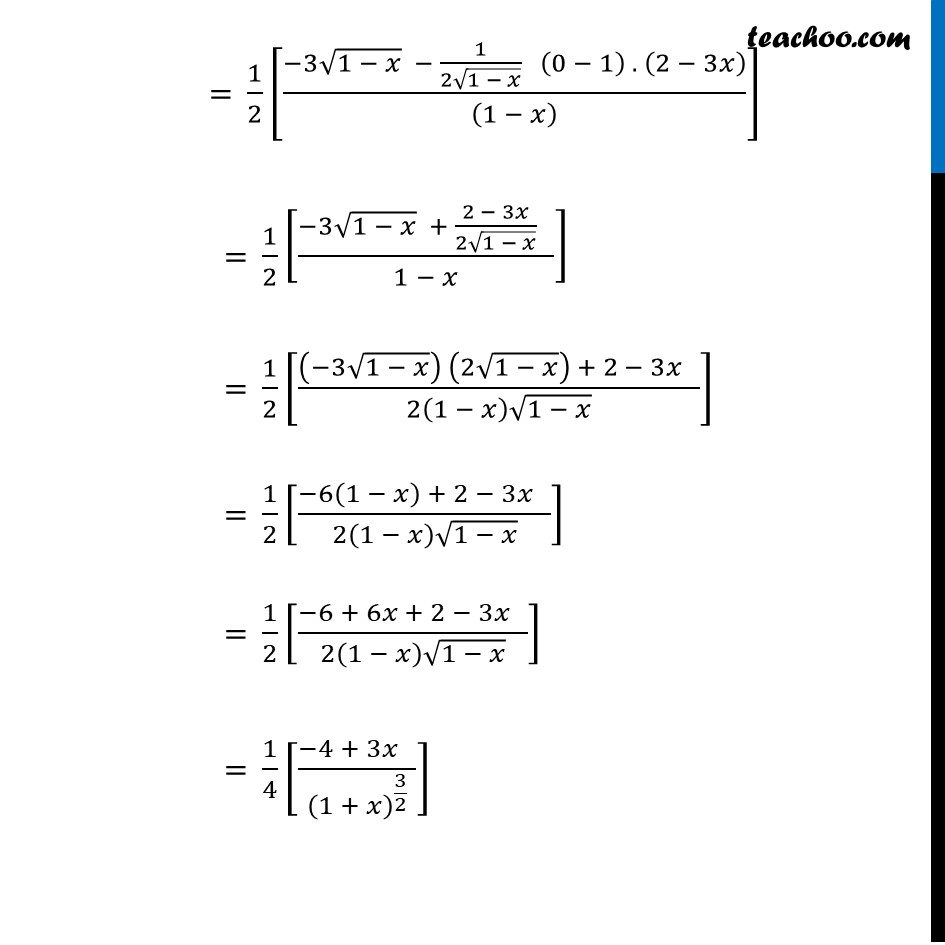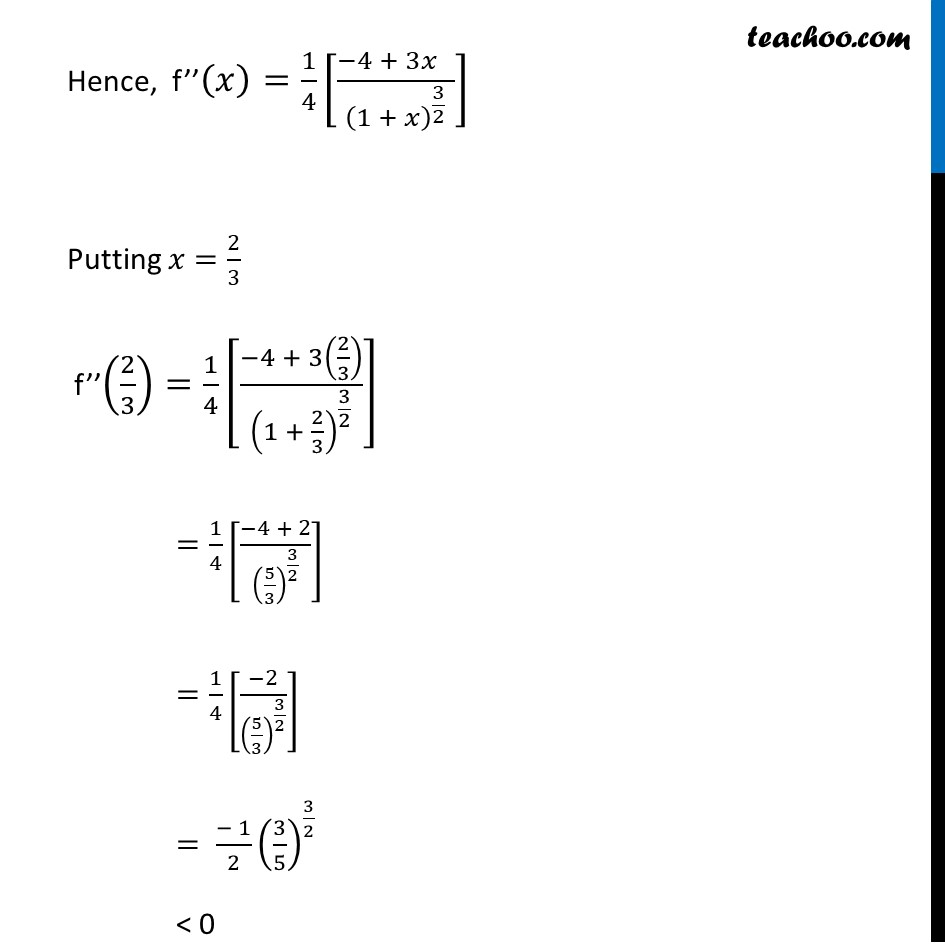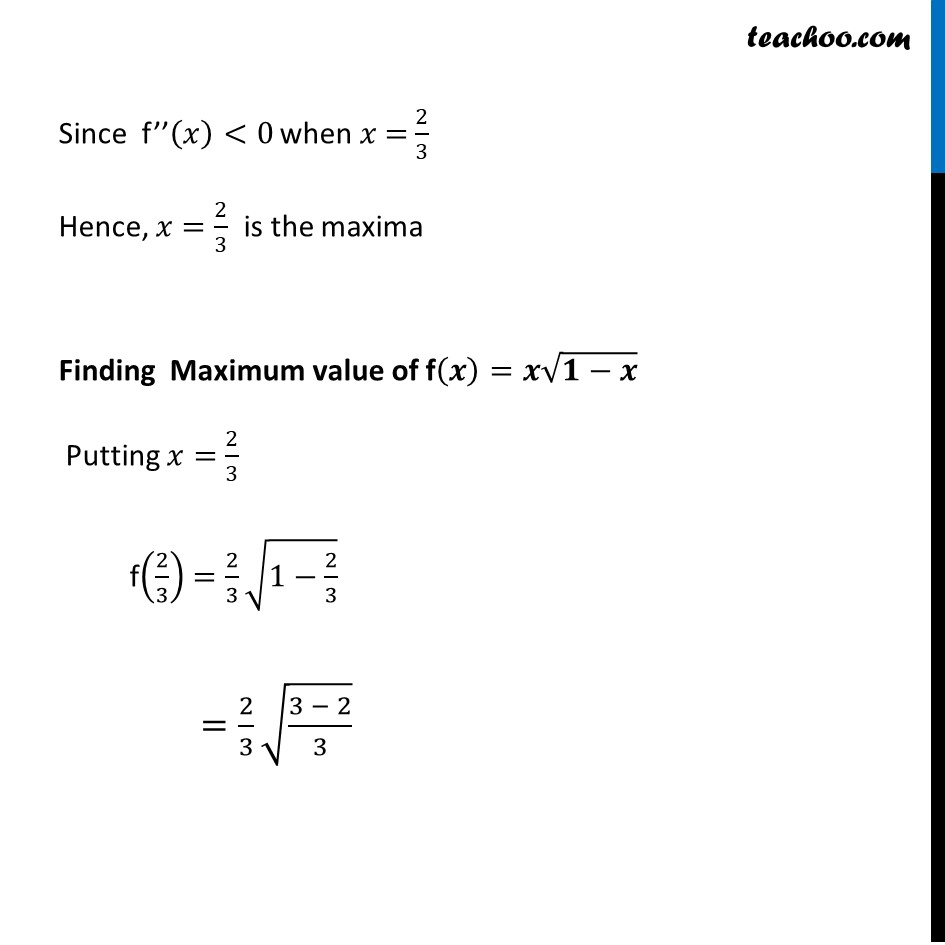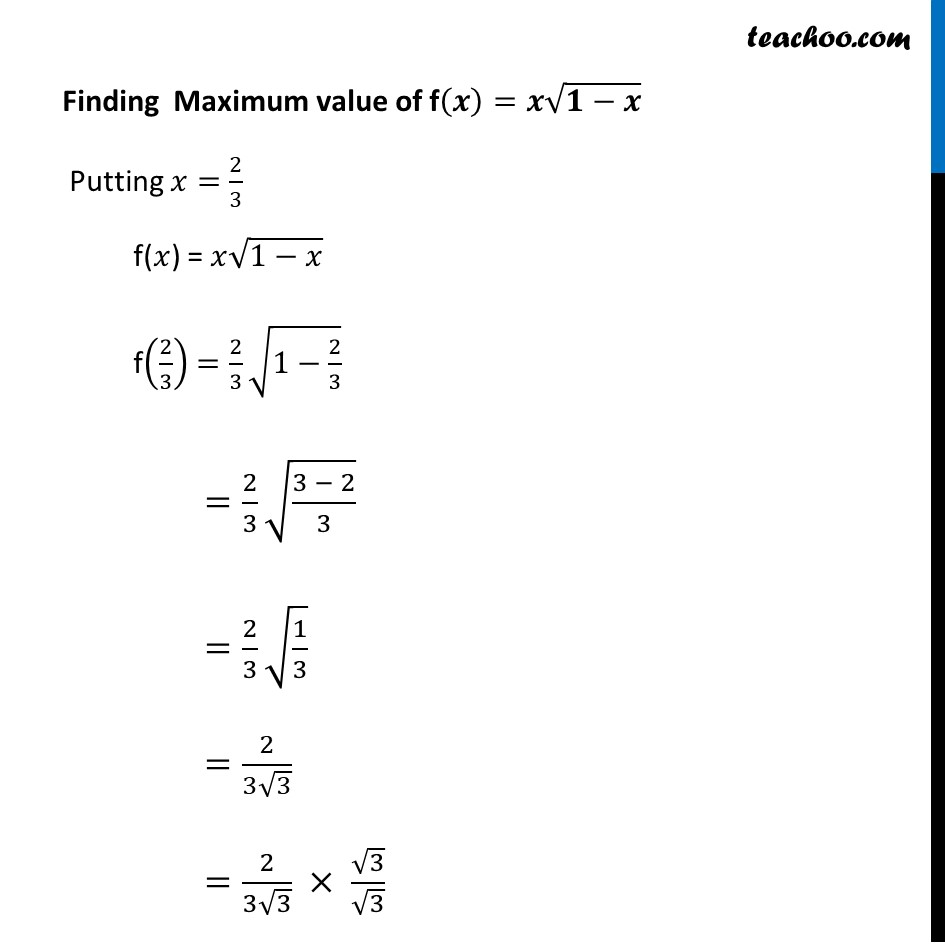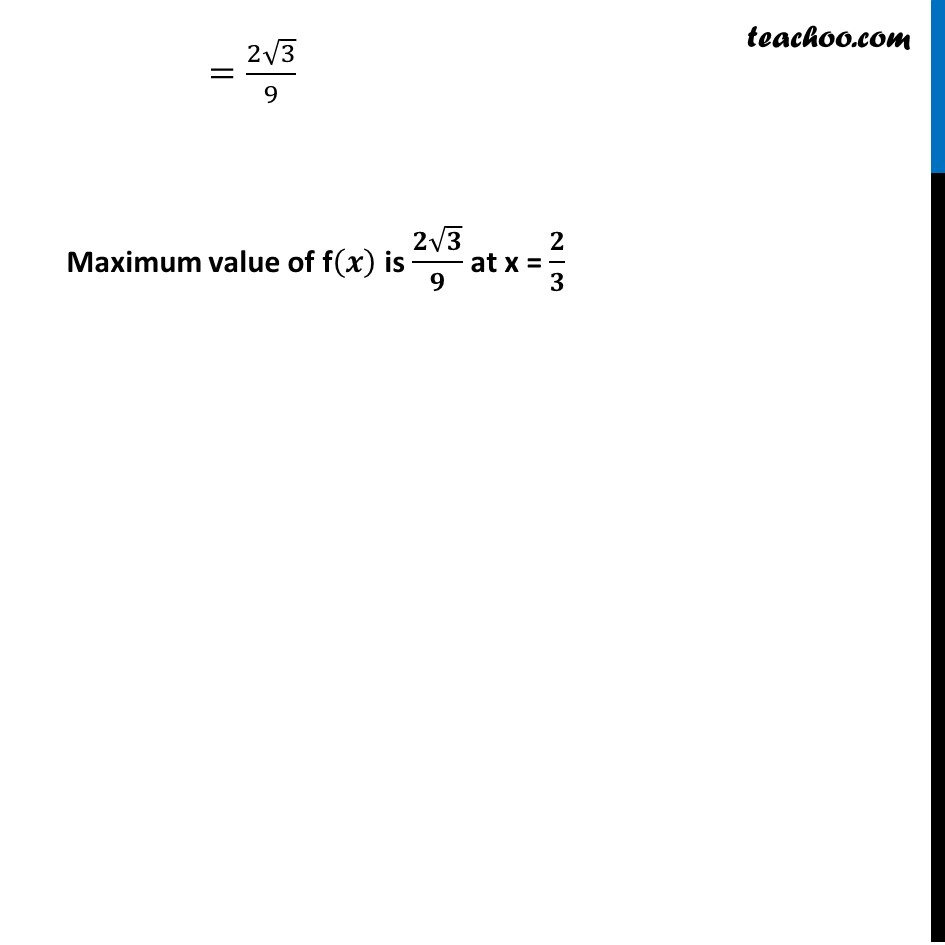1. Chapter 6 Class 12 Application of Derivatives (Term 1)
2. Serial order wise
3. Ex 6.5

Transcript

Ex 6.5, 3 Find the local maxima and local minima, if any, of the following functions. Find also the local maximum & the local minimum values, as the case may be: (i) f (𝑥)=𝑥2 f(𝑥)=𝑥^2 Finding f’(x) f’(x) = 2x Putting f’(x) = 0 2x = 0 x = 0 Finding f’’(x) f’(x) = 2x Differentiating again f’’(x) = 2 Since f’’(x) > 0 for x = 0 So, f(x) is minimum at x = 0 Minimum value of f(x) at x = 0 f(x) = x2 f(0) = 02 = 0 So, Minimum value of f(x) = 0 There is no maximum value Ex 6.5, 3 Find the local maxima and local minima, if any, of the following functions. Find also the local maximum and the local minimum values, as the case may be: (ii) 𝑔(𝑥)=𝑥3 –3𝑥𝑔(𝑥)=𝑥3 –3𝑥 Finding g’(𝒙) g’(𝑥)=𝑑(𝑥^3 − 3𝑥)/𝑑𝑥 g’(𝑥)=3𝑥^2−3 Putting g’(𝒙)=𝟎 3𝑥^2−3=0 3𝑥^2=3 𝑥^2=3/3 𝑥^2=1 𝑥=±1 So, x = 1 & x = –1 Finding g’’(𝒙) g’(𝑥)=3𝑥^2−3 g’’(𝑥)=𝑑(3𝑥^2−3)/𝑑𝑥 = 6𝑥−0 = 6𝑥 Putting 𝒙=𝟏 in g’’(x) g’’(1)=6(1)= 6 > 0 Thus, g’’(𝑥)>0 when 𝑥=1 ⇒ 𝑥=1 is point of local minima & g(𝑥) is minimum at 𝑥=1 Local minimum value g(𝑥)=𝑥^3−3𝑥 g(1)=(1)^3−3(1) =1−3 =−𝟐 Putting 𝒙=−𝟏 in g’’(x) g’’(−1)=6(−1)= –6 < 0 Thus, g’’(𝑥)<0 when 𝑥=−1 ⇒ 𝑥=−1 is point of local maxima & g(𝑥) is maximum at 𝑥=−1 Local minimum value g(𝑥)=𝑥^3−3𝑥 g(−1)=(−1)^3−3(−1) =−1+3 =𝟐 Ex 6.5, 3 Find the local maxima and local minima, if any, of the following functions. Find also the local maximum and the local minimum values, as the case may be: (iii) ℎ(𝑥)=sin⁡𝑥+cos⁡𝑥, 0<𝑥<𝜋/2 ℎ(𝑥)=sin⁡𝑥+cos⁡𝑥, 0<𝑥<𝜋/2 Finding 𝒉′(𝒙) ℎ′(𝑥)=𝑑(sin⁡𝑥 + cos⁡𝑥" " )/𝑑𝑥 ℎ^′ (𝑥)=cos⁡𝑥−sin⁡𝑥 Putting 𝒉′(𝒙)=𝟎 cos⁡𝑥−𝑠𝑖𝑛𝑥=0 cos⁡〖𝑥=𝑠𝑖𝑛 𝑥〗 1 = sin⁡𝑥/cos⁡𝑥 1 = tan 𝑥 tan 𝑥=1 ∴ 𝑥=45°= 𝜋/4 Finding h’’(𝒙) h’(𝑥)=cos⁡𝑥−sin⁡𝑥 h’’(𝑥)=−sin⁡𝑥−cos⁡𝑥 Putting 𝒙=𝝅/𝟒 h’’(π/4)=−sin(π/4)−𝑐𝑜𝑠(π/4) = – 1/√2−1/√2 = (−2)/√2 = – √2 Since h’’(𝑥)<0 when 𝑥=π/4 ∴ 𝑥=π/4 is point of Local Maxima f has Maximum value at 𝒙=𝝅/𝟒 f(𝑥)=𝑠𝑖𝑛𝑥+𝑐𝑜𝑠𝑥 f(π/4)=𝑠𝑖𝑛(π/4)+𝑐𝑜𝑠(π/4) = 1/√2+1/√2 = 2/√2 = √𝟐 Ex 6.5, 3 Find the local maxima and local minima, if any, of the following functions. Find also the local maximum and the local minimum values, as the case may be: (iv) f (𝑥)=sin⁡𝑥 –cos⁡𝑥, 0<𝑥<2 𝜋f (𝑥)=sin⁡𝑥 –cos⁡𝑥, 0<𝑥<2 𝜋 Finding f’(𝒙) f’(𝑥)=cos⁡𝑥−(−sin⁡𝑥 ) f’(𝑥)=cos⁡𝑥+sin⁡𝑥 Putting f’(𝒙)=𝟎 cos⁡𝑥+sin⁡𝑥 = 0 cos⁡𝑥=−sin⁡𝑥 1=(−sin⁡𝑥)/cos⁡𝑥 (−sin⁡𝑥)/cos⁡𝑥 =1 – tan 𝑥=1 tan 𝑥=−1 Since 0 < 𝑥 < 2π & tan 𝑥 is negative tan θ lies in either 2nd or 4th quadrant So, value of 𝑥 is 𝑥=3𝜋/4 𝑜𝑟 7𝜋/4 Now finding f’’(𝑥) f’’(𝑥)=𝑑(cos⁡𝑥 + sin⁡𝑥 )/𝑑𝑥 f’’(𝑥)=−sin⁡𝑥+cos⁡𝑥 Putting 𝒙 = 𝟑𝝅/𝟒 f’’(3𝜋/4)=−𝑠𝑖𝑛(3𝜋/4)+𝑐𝑜𝑠(3𝜋/4) =−𝑠𝑖𝑛(𝜋−𝜋/4)+𝑐𝑜𝑠(𝜋− 𝜋/4) =−𝑠𝑖𝑛(𝜋/4)+(−𝑐𝑜𝑠 𝜋/4) =(−1)/√2−1/√2 =(−2)/√2 =−√2 < 0 Hence f’’(𝑥)<0 when 𝑥 = 3𝜋/4 Thus 𝑥 = 3𝜋/4 is point of local maxima ∴ f(𝑥) is maximum value at 𝑥 = 3𝜋/4 The local maximum value is f(𝑥)=sin⁡𝑥−cos⁡𝑥 f(3𝜋/4)=𝑠𝑖𝑛(3𝜋/4)−𝑐𝑜𝑠(3𝜋/4) =𝑠𝑖𝑛(𝜋−𝜋/4)−𝑐𝑜𝑠(𝜋−𝜋/4) =𝑠𝑖𝑛(𝜋/4)−(−𝑐𝑜𝑠 𝜋/4) As sin (180 − 𝜃) = sin 𝜃 & cos (180 − 𝜃) = −cos 𝜃 =𝑠𝑖𝑛 𝜋/4+𝑐𝑜𝑠 𝜋/4 =1/√2+1/√2 =2/√2 =√2 Now, for 𝒙 = 𝟕𝝅/𝟒 f’’(𝑥)=−sin⁡𝑥+cos⁡𝑥 Putting 𝑥 = 7𝜋/4 f’’(7𝜋/4)=−sin⁡(7𝜋/4)+cos⁡(7𝜋/4) f’’(7𝜋/4)=−𝑠𝑖𝑛(2𝜋−𝜋/4)+𝑐𝑜𝑠(2𝜋−𝜋/4) =−(−𝑠𝑖𝑛(𝜋/4))+𝑐𝑜𝑠(𝜋/4) =𝑠𝑖𝑛 𝜋/4+𝑐𝑜𝑠 𝜋/4 =1/√2 + 1/√2 =2/√2 =√2 > 0 f’’(𝑥)>0 when 𝑥 = 7𝜋/4 Thus 𝑥 = 7𝜋/4 is point of local minima f(𝑥) has minimum value at 𝑥 = 7𝜋/4 As sin (2π − 𝜃) = –sin 𝜃 & cos (2π − 𝜃) = cos 𝜃 Local minimum value is f(𝑥)=𝑠𝑖𝑛𝑥−𝑐𝑜𝑠𝑥 f(7𝜋/4)=𝑠𝑖𝑛(7𝜋/4)−𝑐𝑜𝑠(7𝜋/4) =𝑠𝑖𝑛(2𝜋−𝜋/4)−𝑐𝑜𝑠(2𝜋−𝜋/4) =−𝑠𝑖𝑛(𝜋/4)−𝑐𝑜𝑠(𝜋/4) =(−1)/√2 − 1/√2 =(−2)/√2 =−√2 Thus, f(𝑥) is maximum at x = 𝟑𝝅/𝟒 and maximum value is √𝟐 & f(𝑥) is minimum at x = 𝟕𝝅/𝟒 and maximum value is –√𝟐 Ex 6.5, 3 Find the local maxima and local minima, if any, of the following functions. Find also the local maximum and the local minimum values, as the case may be: (v) 𝑓 (𝑥)=𝑥3 –6𝑥2+9𝑥+15𝑓 (𝑥)=𝑥3 –6𝑥2+9𝑥+15 Finding f’(𝒙) f’(𝑥)=𝑑(𝑥3 – 6𝑥2 + 9𝑥 + 15" " )/𝑑𝑥 f’(𝑥)=3𝑥^2−12𝑥+9 f’(𝑥)=3(𝑥^2−4𝑥+3) Putting f’(𝒙)=𝟎 3(𝑥^2−4𝑥+3)=0 𝑥^2−4𝑥+3=0 𝑥^2−3𝑥−𝑥+3=0 𝑥(𝑥−3)−1(𝑥−3)=0 (𝑥−1)(𝑥−3)=0 So, x = 1 & x = 3 Finding f’’(𝒙) f’(𝑥)=3(𝑥^2−4𝑥+3) f’’(𝑥)=𝑑(3(𝑥^2 − 4𝑥+3))/𝑑𝑥 = 3(2𝑥−4+0) = 6𝑥−12 Putting 𝒙=𝟏 in f’’(𝒙) f’’(1)=6(1)−12 = 6 – 12 = – 6 < 0 Since f’’(𝑥)<0 when 𝑥=1 ⇒ 𝑥=1 is point of local maxima ∴ f(𝑥) is maximum at 𝒙=𝟏 Maximum value of f(𝑥) at 𝑥 = 1 f(𝑥)=𝑥^3−6𝑥^2+9𝑥+15 f(1)=(1)^3−6(1)^2+9(1)+15 = 1 – 6 + 9 + 15 = 19 Putting 𝒙=𝟑 in f’’(x) f’’(𝑥)=6𝑥−12 f’’(3)=6(3)−12 = 18 – 12 = 6 > 0 Since f’’(𝑥)>0 when 𝑥=3 ⇒ 𝑥=3 is point of local minima ∴ f(𝑥) is minimum at 𝒙=𝟑 Minimum value of f(𝑥) at 𝑥 = 3 f(𝑥)=𝑥^3−6𝑥^2+9𝑥+15 f(3)=(3)^3−6(3)^2+9(3)+15 = 27 – 54 + 27 + 15 = 15 Ex 6.5, 3 Find the local maxima and local minima, if any, of the following functions. Find also the local maximum and the local minimum values, as the case may be: (vi) g (𝑥) = 𝑥/2 + 2/𝑥 , 𝑥 > 0g (𝑥) = 𝑥/2 + 2/𝑥, 𝑥 > 0 Finding g’(𝒙) g’(𝑥)=𝑑/𝑑𝑥 (𝑥/2+2/𝑥) =𝑑/𝑑𝑥 (𝑥/2)+𝑑/𝑑𝑥 (2𝑥^(−1) ) =1/2−2𝑥^(−2) =1/2−2/𝑥^2 Putting g’(𝒙)=𝟎 1/2−2/𝑥^2 =0 (𝑥^2− 4)/(2𝑥^2 )=0 𝑥^2−4=0 ×2𝑥^2 (𝑥−2)(𝑥+2)=0 So, 𝑥=2 & 𝑥=−2 Since 𝑥>0 is given, we consider only 𝑥=2 Finding g’’(𝒙) g’(𝑥)=1/2−2/𝑥^2 g’’(𝑥)=𝑑/𝑑𝑥 (1/2−2/𝑥^2 ) = 0 – 2 . (−2) 𝑥^(−2−1) = 4𝑥^(−3) = 4/𝑥^3 Putting value 𝑥=2 in g’’(𝑥) g’’(𝑥)=4/(2)^3 = 4/8= 1/2 Since g’’ (x) > 0, for x = 2 g(x) is minimum at x = 2 Minimum value of g(𝑥) is g(𝑥)=𝑥/2+2/𝑥 Putting 𝑥=2 g(2)= 2/2+2/2 = 1 + 1 = 2 Ex 6.5, 3 Find the local maxima and local minima, if any, of the following functions. Find also the local maximum and the local minimum values, as the case may be: (vii) g (𝑥) = 1/(𝑥^2 + 2)Finding g’(𝒙) g’(𝑥)=𝑑/𝑑𝑥 (1/(𝑥^2 + 2)) g’(𝑥)=(𝑑(𝑥^2 + 2)^(−1))/𝑑𝑥 g’(𝑥)=−1(𝑥^2+2)^(−1−1) × (2𝑥+0) g’(𝑥)=−2𝑥(𝑥^2+2)^(−2) g′(𝑥)=( −2𝑥 )/(𝑥^2 + 2)^2 Putting g’(𝒙)=𝟎 ( −2𝑥 )/(𝑥^2+2)^2 =0 –2𝑥=0 ×(𝑥^2+2)^2 –2𝑥=0 𝑥=0 Finding g’’(𝒙) g’(𝑥)=(−2𝑥)/(𝑥^2 + 2)^2 g’’(𝑥)=(𝑑(−2𝑥)/𝑑𝑥 . 〖 (𝑥^2 + 2)〗^2 − (𝑑(𝑥^2 + 2)^2)/𝑑𝑥 . (−2𝑥))/((𝑥^2 + 2)^2 )^2 Using quotient rule as (𝑢/𝑣)^′=(𝑢^′ 𝑣 − 𝑣^′ 𝑢)/𝑣^2 =(−2 (𝑥^2 + 2)^2−2 (𝑥^2 + 2)^(2−1).𝑑(𝑥^2 + 2)/𝑑𝑥 . (−2𝑥))/((𝑥^2 + 2)^2 )^2 =(−2 (𝑥^2 + 2)^2−2 (𝑥^2 + 2)(2𝑥 + 0) (−2𝑥))/(𝑥^2 + 2)^4 =(−2 (𝑥^2 + 2)^2−2 (𝑥^2 + 2)(2𝑥) (−2𝑥))/(𝑥^(2 )+ 2)^4 =(−2 (𝑥^2 + 2)^2+ 8𝑥^2 (𝑥^2 + 2))/(𝑥^(2 )+ 2)^4 =(−2 (𝑥^2 + 2)[(𝑥^(2 )+ 2) − 4𝑥^2 ])/(𝑥^2 + 2)^4 =(−2 (𝑥^2 + 2)(−3𝑥^2 + 2))/(𝑥^2 + 2)^4 =(−2(−3𝑥^2 + 2))/(𝑥^(2 )+ 2)^3 Putting x = 0 in g’’(x) g’’(0)=(−2(−3(0) + 2))/(0^2 + 2)^3 =(−2(0 + 2))/(2)^3 =(−4)/8=(−1)/2 Hence g’’(𝑥)<0 when 𝑥 = 0 ∴ 𝑥 = 0 is point of local maxima Thus, g(𝑥) is maximum at 𝒙 = 0 Maximum value of g(𝒙) at x = 0 g(𝑥)=1/(𝑥^(2 )+ 2) g(0)=1/(0^2 + 2) = 1/2 Maximum value is 𝟏/𝟐 Ex 6.5, 3 Find the local maxima and local minima, if any, of the following functions. Find also the local maximum and the local minimum values, as the case may be: (viii) f(𝑥) = 𝑥√(1−𝑥), 𝑥 > 0Finding f’(𝒙) f’(𝑥)=𝑑(𝑥√(1 − 𝑥))/𝑑𝑥 f’(𝑥)=𝑑(𝑥)/𝑑𝑥 . √(1−𝑥) + 𝑑(√(1 − 𝑥))/𝑑𝑥 . 𝑥 = 1 . √(1−𝑥) + 1/(2√(1 − 𝑥)) . 𝑑(1 − 𝑥)/𝑑𝑥 . 𝑥 = √(1−𝑥) + 1/(2√(1 − 𝑥)) (0 −1) . 𝑥 = √(1−𝑥) – 𝑥/(2√(1 − 𝑥)) = (2(√(1 − 𝑥) )^2− 𝑥)/(2√(1 − 𝑥)) = (2(1 − 𝑥) − 𝑥)/(2√(1 − 𝑥)) = (2 − 2𝑥 − 𝑥)/(2√(1 − 𝑥)) = (2 − 3𝑥)/(2√(1 − 𝑥)) Putting f’(𝒙)=𝟎 (2 − 3𝑥)/(2√(1 − 𝑥))=0 2 – 3𝑥 = 0 × 2√(1−𝑥) 2 – 3𝑥=0 – 3𝑥=−2 𝑥 =2/3 Finding f’’(𝒙) f’(𝑥)=(2 − 3𝑥)/(2√(1 − 𝑥)) f’’(𝑥)=𝑑/𝑑𝑥 ((2 − 3𝑥)/(2√(1 − 𝑥))) = 1/2 [(𝑑(2 − 3𝑥)/𝑑𝑥 . √(1 − 𝑥) − 𝑑(√(1 − 𝑥))/𝑑𝑥 . (2 − 3𝑥))/(√(1 − 𝑥))^2 ] = 1/2 [((0 − 3) √(1 − 𝑥) − 1/(2√(1 − 𝑥)) . 𝑑(1 − 𝑥)/𝑑𝑥 . (2 − 3𝑥))/((1 − 𝑥) )] Using quotient rule as (𝑢/𝑣)^′=(𝑢^′ 𝑣 − 𝑣^′ 𝑢)/𝑣^2 = 1/2 [(−3√(1 − 𝑥) − 1/(2√(1 − 𝑥)) (0 − 1) . (2 − 3𝑥))/((1 − 𝑥) )] = 1/2 [(−3√(1 − 𝑥) + (2 − 3𝑥)/(2√(1 − 𝑥)) )/(1 − 𝑥)] = 1/2 [((−3√(1 − 𝑥)) (2√(1 − 𝑥)) + 2 − 3𝑥 )/(2(1 − 𝑥) √(1 − 𝑥))] = 1/2 [(−6(1 − 𝑥) + 2 − 3𝑥 )/(2(1 − 𝑥) √(1 − 𝑥))] = 1/2 [(−6 + 6𝑥 + 2 − 3𝑥 )/(2(1 − 𝑥) √(1 − 𝑥))] = 1/4 [(−4 + 3𝑥 )/(1 + 𝑥)^(3/2) ] Hence, f’’(𝑥)=1/4 [(−4 + 3𝑥 )/(1 + 𝑥)^(3/2) ] Putting 𝑥=2/3 f’’(2/3)=1/4 [(−4 + 3(2/3))/(1 + 2/3)^(3/2) ] =1/4 [(−4 + 2)/(5/3)^(3/2) ] =1/4 [(−2)/(5/3)^(3/2) ] = (− 1)/2 (3/5)^(3/2) < 0 Since f’’(𝑥)<0 when 𝑥 = 2/3 Hence, 𝑥=2/3 is the maxima Finding Maximum value of f(𝒙)=𝒙√(𝟏−𝒙) Putting 𝑥=2/3 f(2/3)=2/3 √(1−2/3) =2/3 √((3 − 2)/3) Finding Maximum value of f(𝒙)=𝒙√(𝟏−𝒙) Putting 𝑥=2/3 f(𝑥) = 𝑥√(1−𝑥) f(2/3)=2/3 √(1−2/3) =2/3 √((3 − 2)/3) =2/3 √(1/3) =2/(3√3) =2/(3√3) × √3/√3 =(2√3)/9 Maximum value of f(𝒙) is (𝟐√𝟑)/𝟗 at x = 𝟐/𝟑

Ex 6.5